• Turing Machine 图灵机 下推自动机类似，都有七个部分，不过栈的符号集合转变为taple集合，转移函数对taple进行操作，并且b是一个空字符， 瞬时表如下 图灵机接受的语言叫递归...以上为第三章内容，主要讲解了图...
Turing Machine
图灵机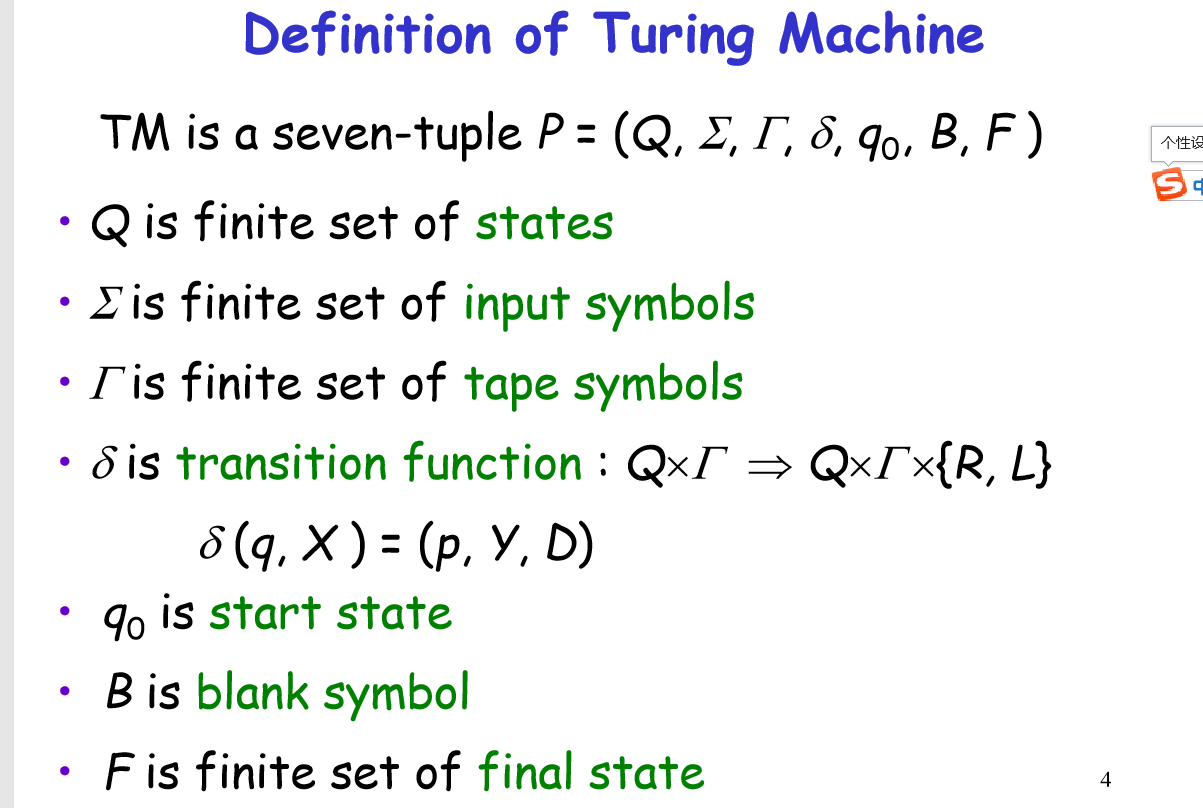与下推自动机类似，都有七个部分，不过栈的符号集合转变为taple集合，转移函数对taple进行操作，并且b是一个空字符，
瞬时表如下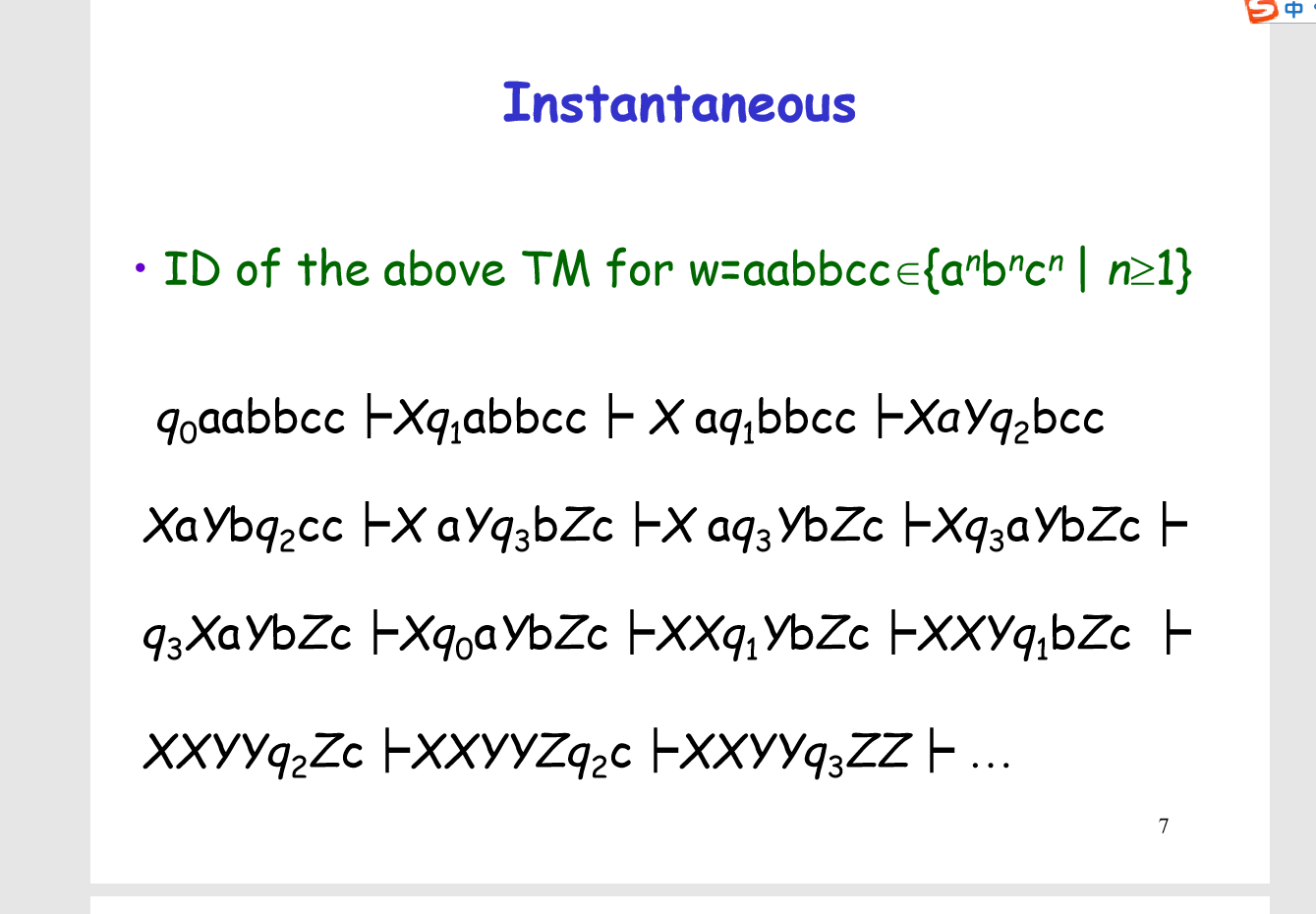图灵机接受的语言叫递归可枚举语言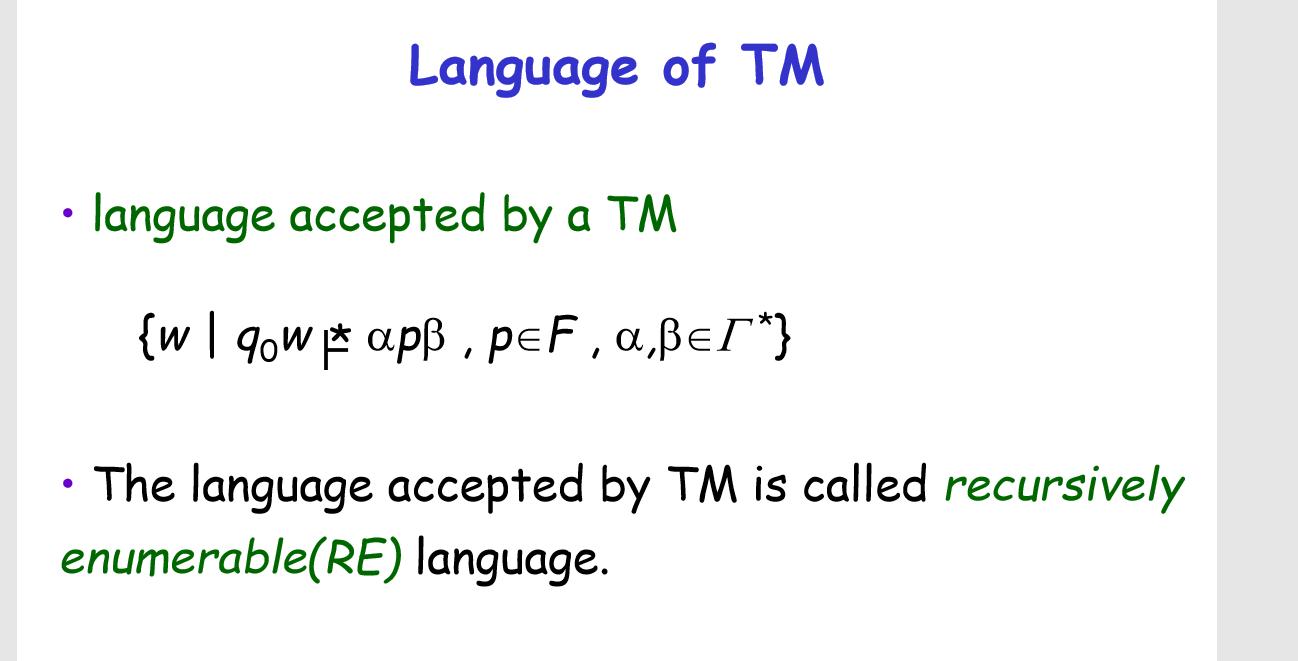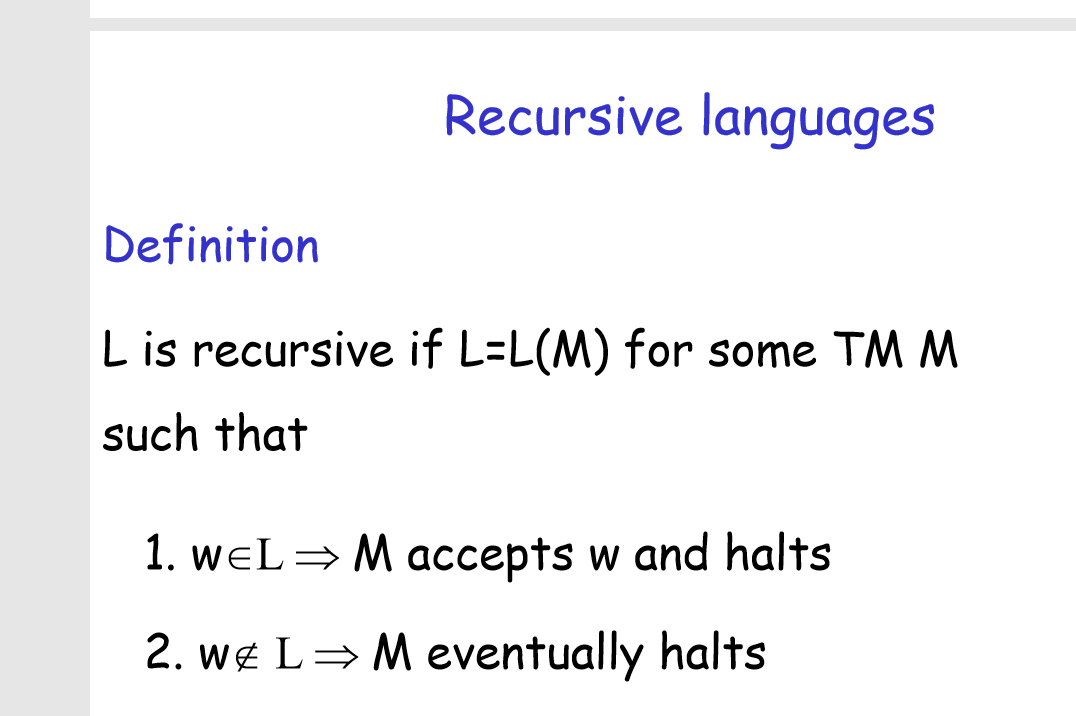以上为递归语言的定义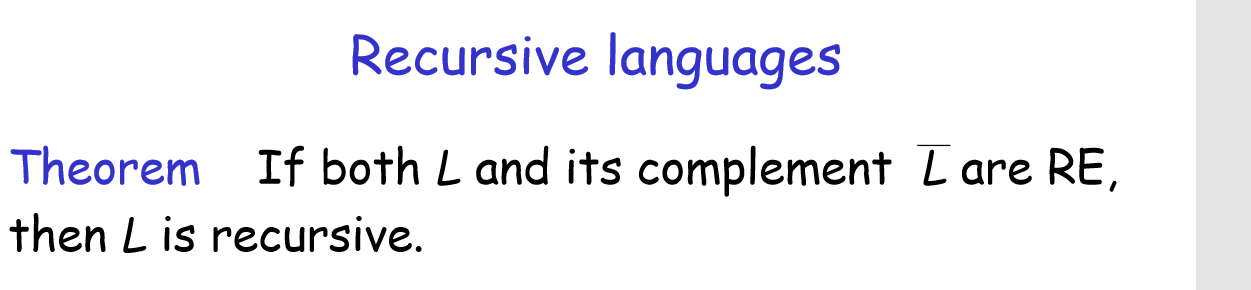递归语言的性质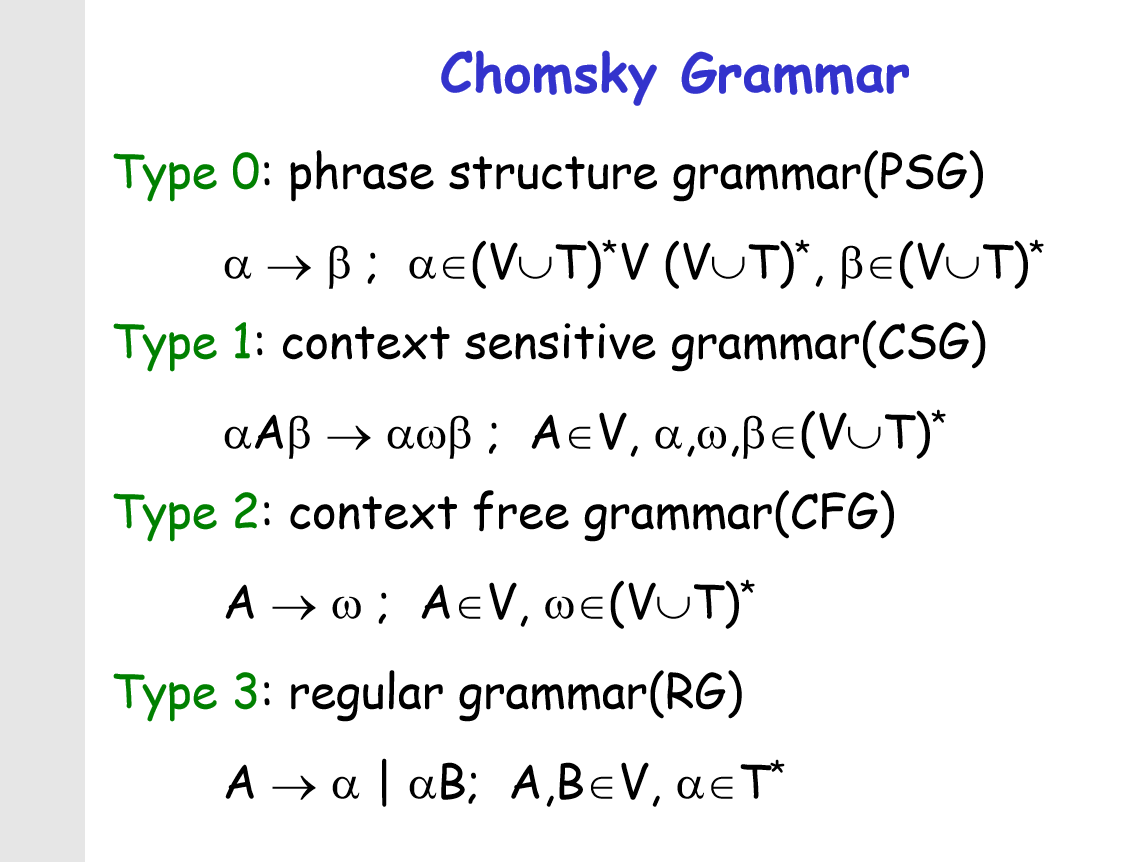乔布斯及语法的四种类型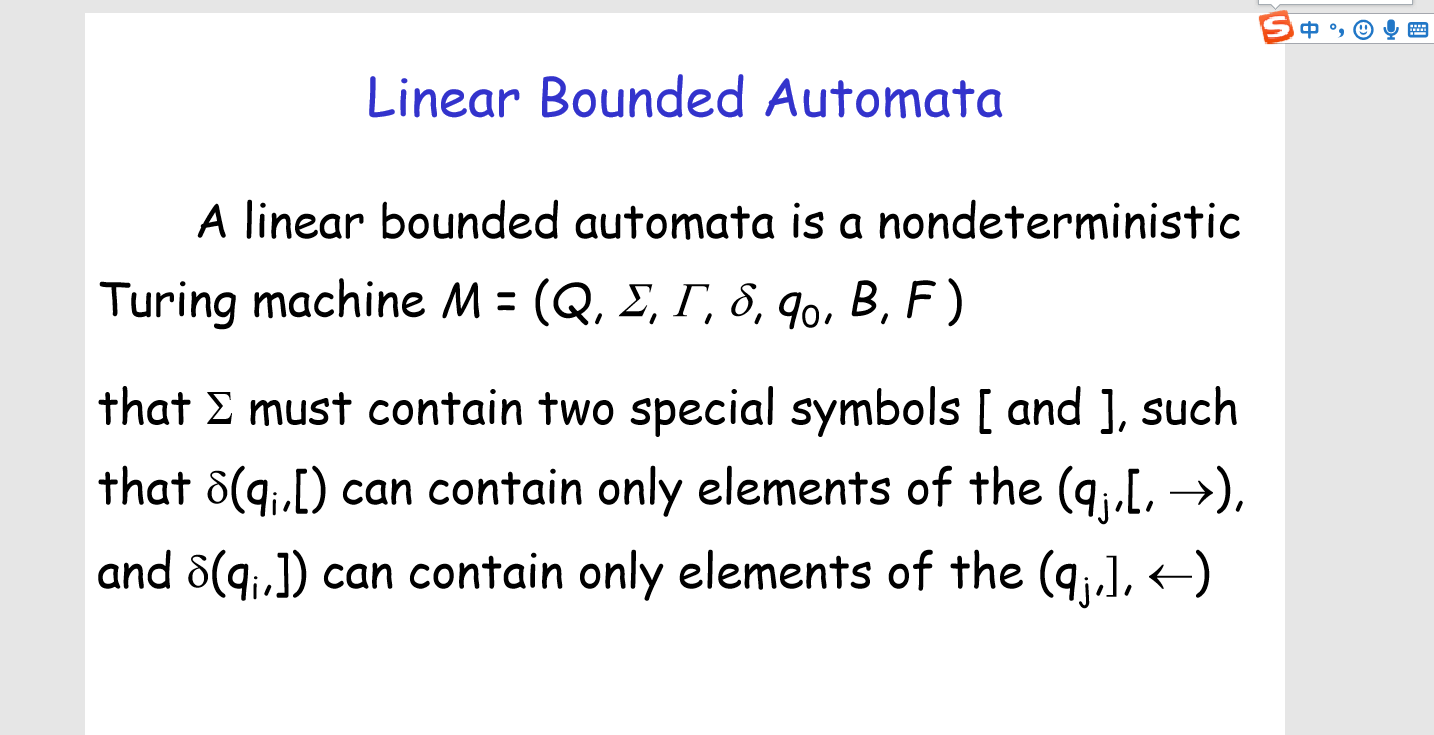线程绑定的自动机，非确定性图灵机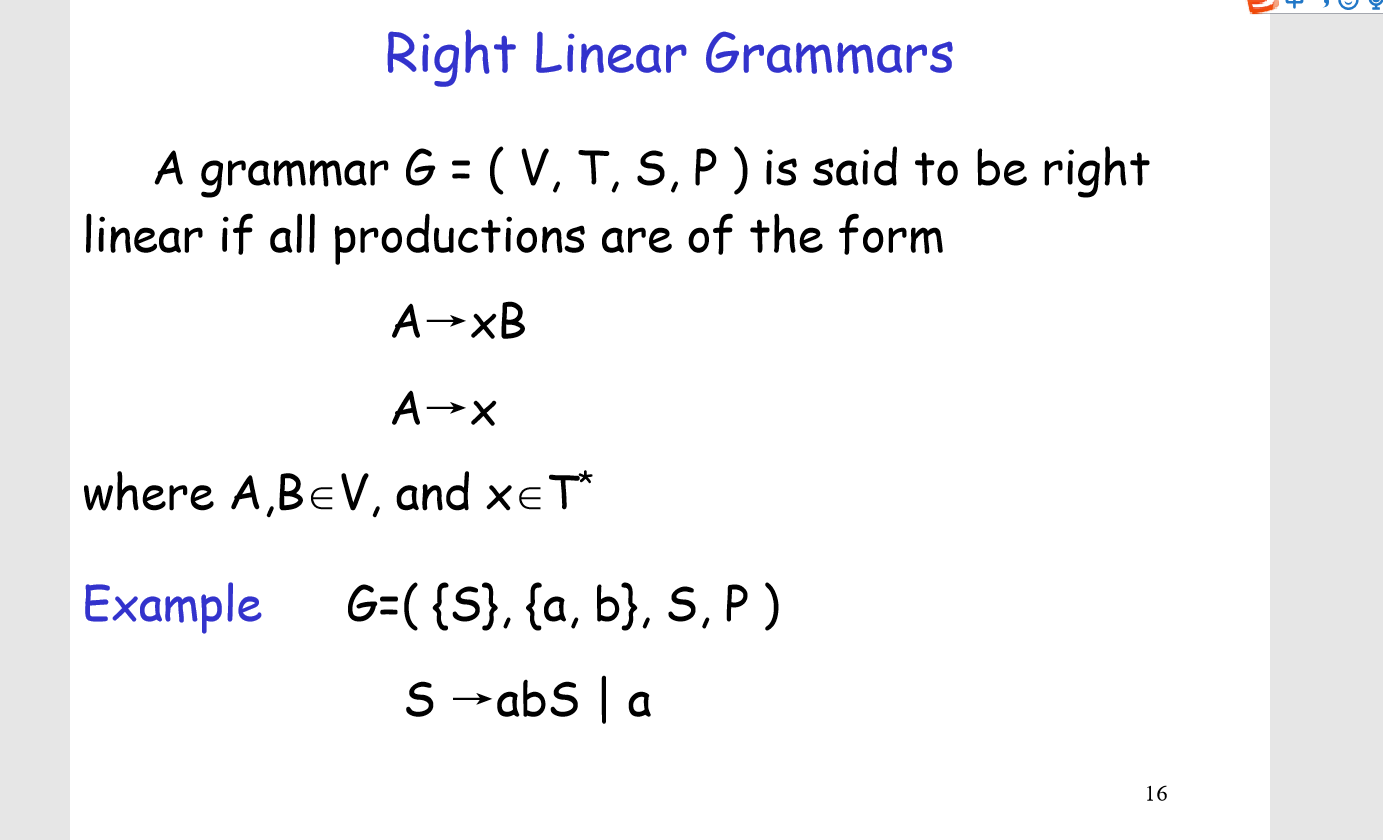右线程语法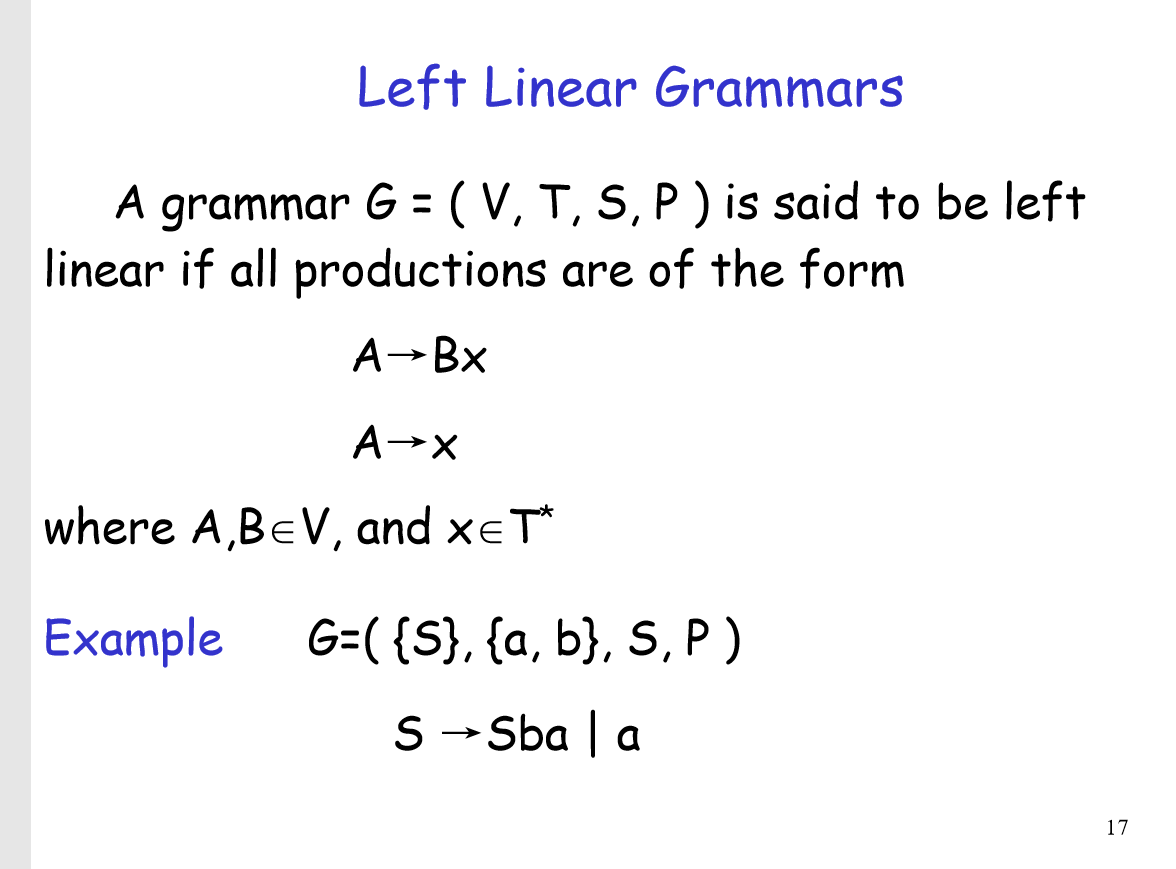左线程语法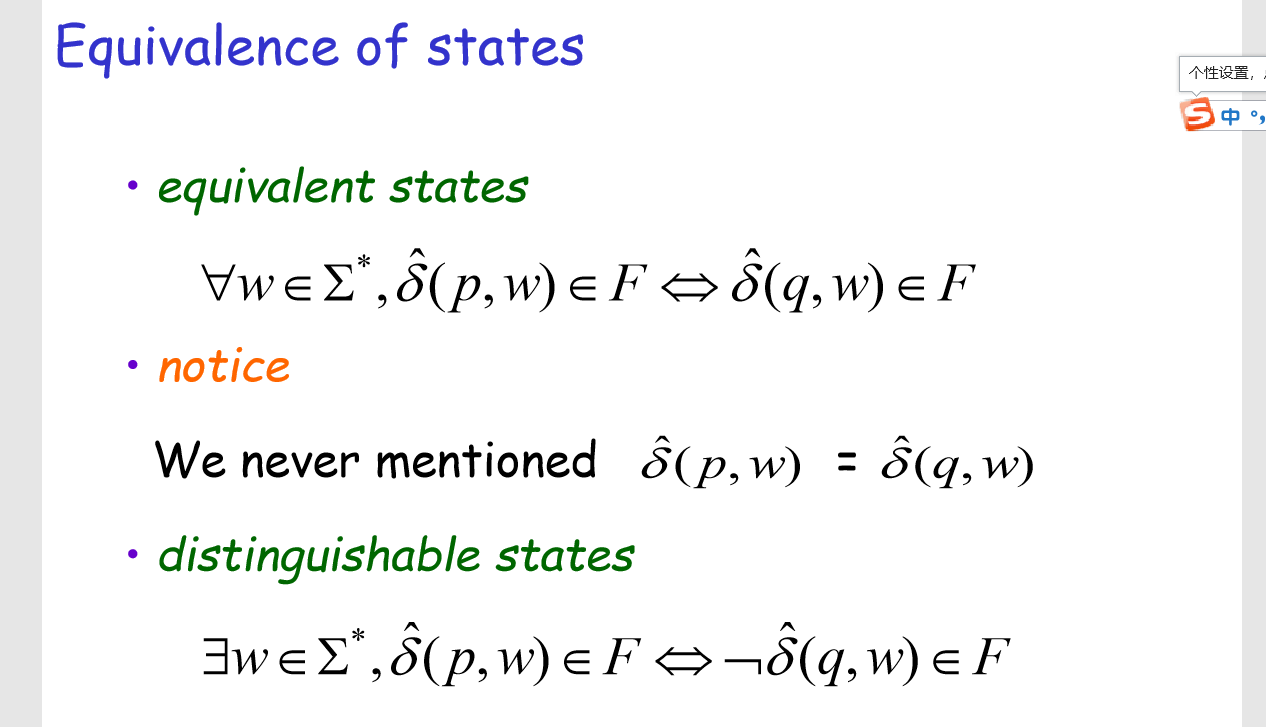等价状态和可区分状态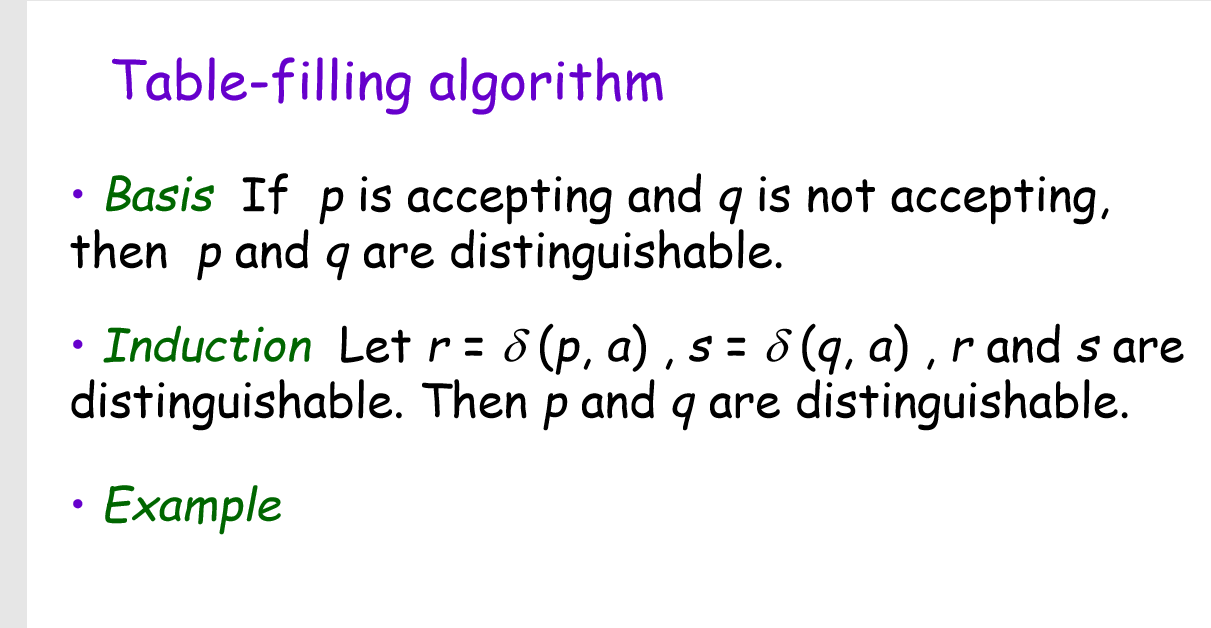以上为第三章内容，主要讲解了图灵机以及递归可枚举语言的使用。


展开全文• 考点：语言⇒DFA（设计DFA） 解：（1）M=({q0,q1,q2,q3},{a,b},δ,q0,{q3})M=(\{q_0,q_1,q_2,q_3\},\{a,b\},δ,q_0,\{q_3\})M=({q0​,q1​,q2​,q3​},{a,b},δ,q0​,{q3​})，其中 δ 如下： （2）M=({q0,q1,q2},{...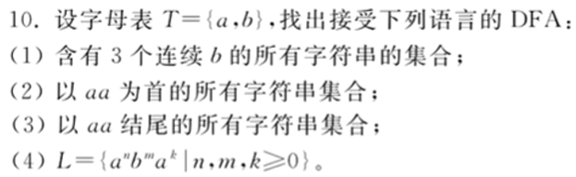考点：语言⇒DFA（设计DFA，记录关键信息）
解：（1）$M=(\{q_0,q_1,q_2,q_3\},\{a,b\},δ,q_0,\{q_3\})$，其中 δ 如下：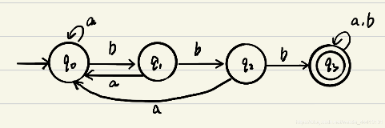（2）$M=(\{q_0,q_1,q_2\},\{a,b\},δ,q_0,\{q_2\})$，其中 δ 如下：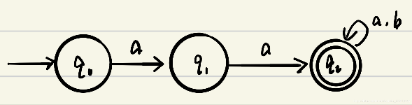（3）$M=(\{q_0,q_1,q_2\},\{a,b\},δ,q_0,\{q_2\})$，其中 δ 如下：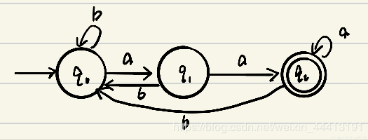（4）$M=(\{q_0,q_1,q_2\},\{a,b\},δ,q_0,\{q_0,q_1,q_2\})$，其中 δ 如下：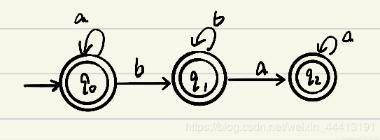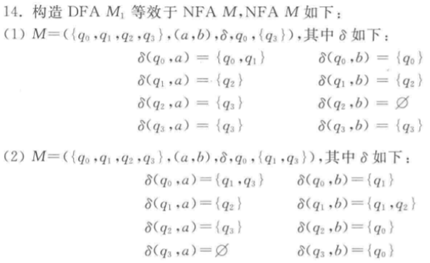考点：NFA⇒DFA （子集构造法：始态出发，遇什么填什么）
解：（1）$DFA-M_1=(Q_1,\{a,b\},δ_1,\{q_0\},\{ \{q_0,q_1,q_3 \},\{q_0,q_2,q_3 \},\{q_0,q_3 \},\{q_0,q_1,q_2,q_3\})，其中 Q_1=\{\{q_0\},\{q_0,q_1\},\{q_0,q_1,q_2\},\{q_0,q_2\},\{q_0,q_1,q_2,q_3\},\{q_0,q_1,q_3\},\{q_0,q_2,q_3\},\{q_0,q_3\}\}$
$δ_1$ 满足: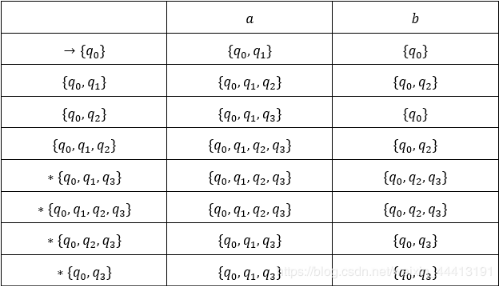（2）$DFA M_1=(\{Q_1,\{a,b\},δ_1,\{q_0\},\{\{q_1\},\{q_3\},\{q_1,q_2\},\{q_0,q_1,q_2\},\{q_1,q_3\},\{q_1,q_2,q_3\},\{q_2,q_3\})$，其中 $Q_1=\{ \{q_0\},\{q_1,q_3\},\{q_1\},\{q_2\},\{q_0,q_1,q_2\},\{q_1,q_2\},\{q_3\},\{q_1,q_2,q_3\},\{q_2,q_3\}\}$
$δ_1$ 满足: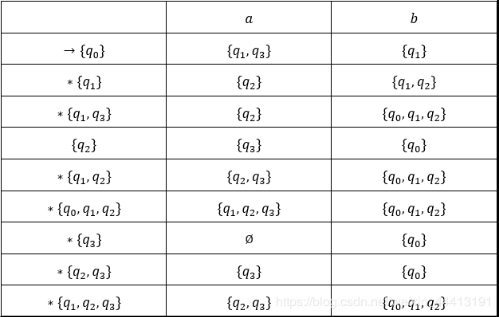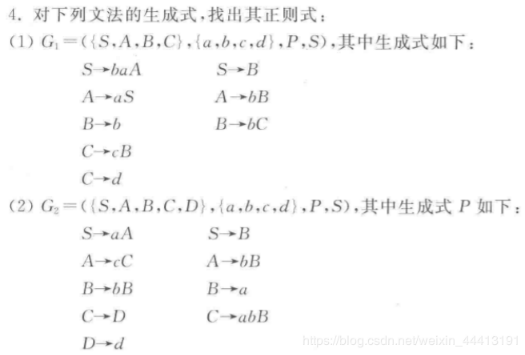考点：文法⇒正则式 （R规则：设x→αx+β（x→αx|β），则x=α*β）
解：（1）联立方程组：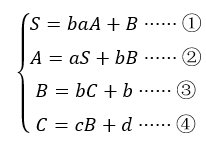将④带入③中得：$B=bcB+bd+b$，利用规则R，得 $B=(bc)^* (bd+b)····⑤$
再将②和⑤带入①得：$S=baaS+babB+B$
$=baaS+(bab+ε)B$
$=baaS+(bab+ε) (bc)^* (bd+b)$
$=(baa)^* (bab+ε) (bc)^* (bd+b)$
∴得到正则式为 $(baa)^* (bab+ε) (bc)^* (bd+b)$
（2）联立方程组：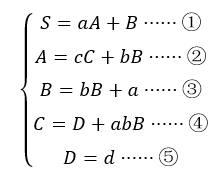由③和规则R得：$B=b^* a······⑥$
将⑤⑥带入④中得：$C=d+abb^* a=d+ab^+ a······⑦$
将⑥⑦带入②中得：$A=c(d+ab^+ a)+b^+ a······⑧$
将⑥⑧带入①中得：$S=ac(d+ab^+ a)+ab^+ a+b^* a$
$=acd+acab^+ a+ab^+ a+b^* a$
∴得到正则式为 $acd+acab^+ a+ab^+ a+b^* a$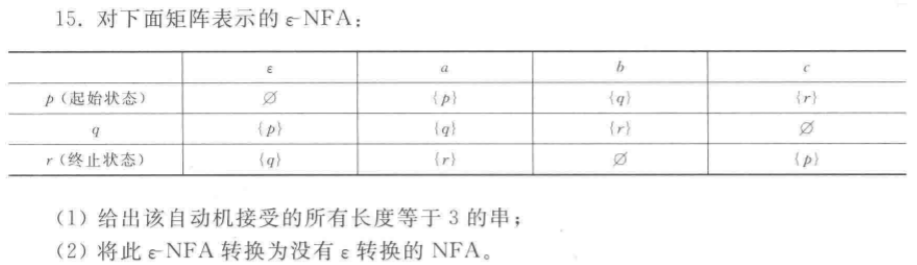考点：自动机接受的语言；ε-NFA⇒NFA （先判断F，每步扩展空闭包）
解：（1）矩阵对应的ε-NFA如下：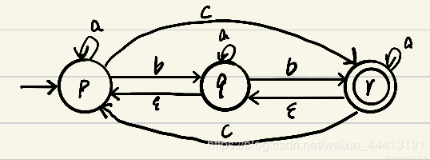利用排除法有，共23个串：$aac,abb,abc,aca,acb,acc,bab,bac,bba,bbb,bbc,bca,bcb, bcc,caa,cab,cac,cba,cbb,cbc,cca,ccb,ccc$
（2）因为$eclosure(q0)∩F=Ø$，则$F_1=F=\{r\}$，则$NFA -M=(\{p,q,r\},\{a,b,c\},δ_1,p,\{r\})$，其中$δ_1$：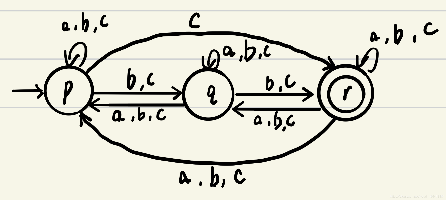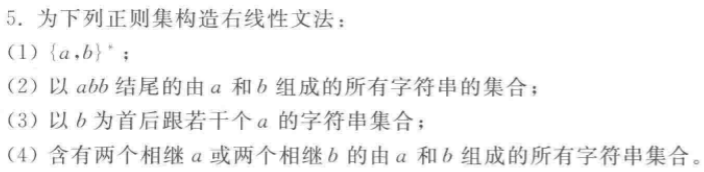考点：正则集⇒右线性文法 （R规则逆用）
解：（2）题目对应的正则表达式为$(a+b)^* abb$，逆用R规则得到对应的右线性文法为 $G=(\{S\},\{a,b\},P,S)$，其中P：$S→(a+b)S|abb$，即 $S→aS|bS|abb$
（4）题目对应的正则表达式为 $(a+b)^* (aa+bb)(a+b)^*$，逆用R规则得到对应的右线性文法为 $G=(\{S,A\},\{a,b\},P,S)$，其中P为 $S→(a+b)S|aaA|bbA, A→(a+b)A|ε$，即 $S→aS|bS|aaA|bbA, A→aA|bA|ε$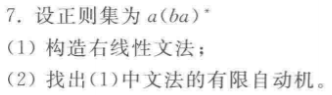考点：正则集⇒右线性文法；正则集⇒ε-NAF（有限自动机）；右线性文法⇒NFA
解：（1）逆用R规则得到对应右线性文法 $G=(\{S,A\},\{a,b\},P,S)$，其中P为 $S→aA,A→baA|ε$
（2）由（1）中右线性文法有 $S→ε∉P$，则转换为 $DFA-M=(\{S,A,H\},\{a,b\},δ,S,\{H\})$，其中$δ$为：
对于产生式 $S→aA$，有 $δ(S,a)=\{A\}$
对于产生式 $A→bS$，有 $δ(A,b)=\{S\}$
对于产生式 $A→ε$，有 $δ(A,ε)=\{H\}$
状态转移图如下: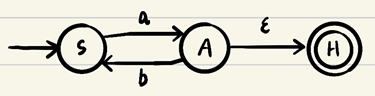继续进行化简，消去 $ε$，将状态A与H合并，得到下图：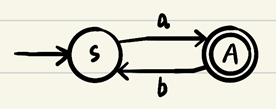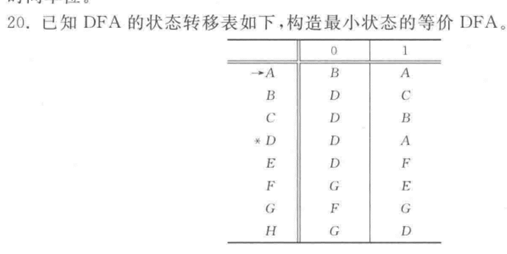考点：DFA⇒最小状态DFA （填表法）
解：$E,F,G,H$ 为不可达状态，删去后利用填表法：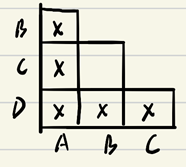由表格得： B,C等价，$\{B,C\}$用 $[B]$ 表示，则等价最小DFA的转移图为：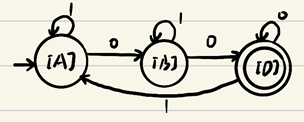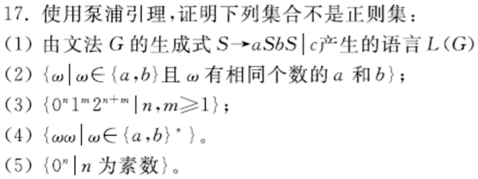考点：正则集的泵浦引理
解：（1）L(G)中，a和b的个数相等。由泵浦引理，假设L是正则集，取足够大的整数 k，$ω=a^k (cb)^k c，|ω|=3k+1>k,|ω_0|>0，0<|ω_1ω_0|≤k$，则 $ω_0$ 只能处于 $a^k$ 段。当 $i≠1$时，a的个数发生改变，而b的个数不会变，a的个数≠b的个数，因此与假设矛盾，则L(G)不是正则集。
（3）由泵浦原理，假设L是正则集，取足够大的整数 $k,ω=0^k 12^{k+1},|ω|=2k+2>k,|ω_0 |>0，0<|ω_1 ω_0 |≤k$，限制了 $ω_0$ 只能在 $0^k$ 段。当 $i=0$ 时，$ω_1 ω_0^0 ω_2$中0的数量减少，而1和2的个数没变，不满足 $0^n 1^m 2^{n+m}$。与假设矛盾，L不是正则集。
（4）由泵浦原理，假设L是正则集，取足够大的整数 $k，ω=a^k ba^k b，|ω|=2k+2>k，|ω_0 |>0，0<|ω_1 ω_0 |≤k$，限制了 $ω_0$ 只能处于 $a^k$ 段。当 $i≠0$ 时，$a^k$ 段中a的个数发生改变，不等于k，不满足 ωω 的形式。与假设矛盾，L不是正则集。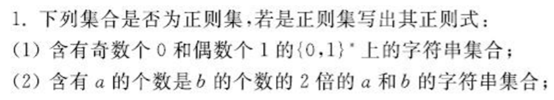考点：正则集的泵浦引理；设计正则式
解：（1）首先根据题意设计DFA，0、1的奇偶性需要4个状态来记录，因此容易得出DFA如下：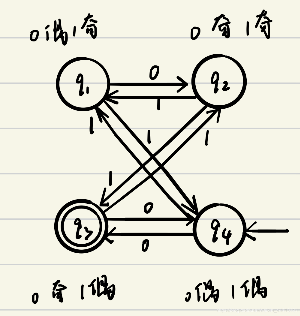再利用状态消去的形式化方法，将DFA转换为正则式：
首先加初态s和终态a，与原自动机相，扩展为广义自动机：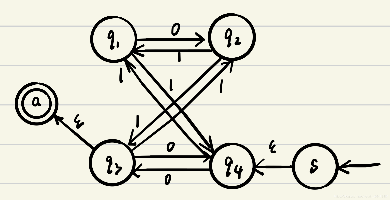接下来逐个消去中间状态，消去 $q_1$：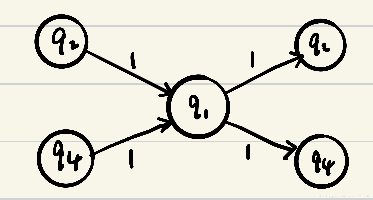得到自动机：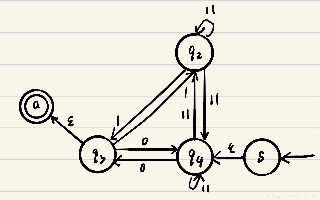最终得到正则式为 $0+(11)^+ 0+(10+(11)^+ 10)(01(11)^* 10)^* (1+01(11)^* 0)(0(11)^* 0+(1+0(11)^* 10)(01(11)^* 10)^* (1+01(11)^* 0))^*$
（2）由泵浦引理，假设L为正则集，取 $ω=a^{2p} b^p,|ω|=|ω_1ω_0ω_2|=3p＞p,0＜|ω_1ω_0|≤p$，因此限制了 $ω_1ω_0$ 只能在 $a^{2p}$ 段，当 $i=0$ 时，a部分的长度减小，但b部分长度不变，a个数≠b个数，不符合L，与假设矛盾，因此L不是正则集。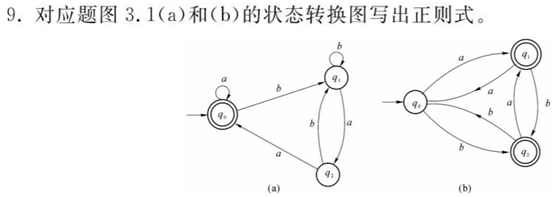考点：有限自动机⇒正则式 （状态消去形式化方法）
解：（a）使用状态消去形式化方法，消去 $q_1$,得到状态图：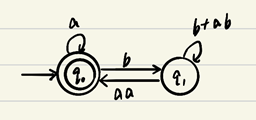直接得到正则式为 $(a+b(b+ab)^* aa)^*$
（b）用形式化消去法，扩展为广义自动机有：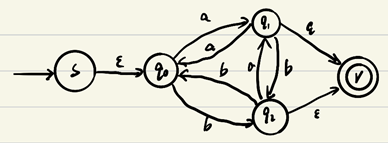消去状态 $q_0$ 有：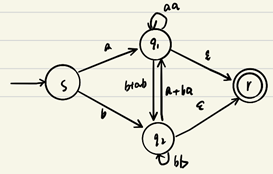消去状态 $q_1$ 有：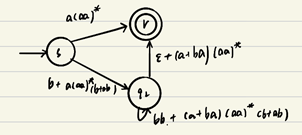由此可直接写出正则式：$a(aa)^*+(b+a(aa)^* (b+ab)) (bb+(a+ba) (aa)^* (b+ab))^* (ε+(a+ba)(aa)^*)$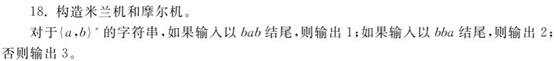考点：构造米兰机和摩尔机；米兰机与摩尔机的相互转换
解：构造摩尔机 $M_1=(\{q_1,q_2,q_3,q_4,q_5\},\{a,b\},\{1,2,3\},δ,g,q_0)$，其中 $δ,g$ 如下：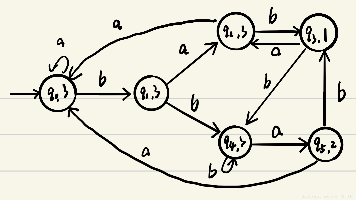直接将摩尔机转换为米兰机有（将状态中的输出放到弧上）$M_2=(\{q_1,q_2,q_3,q_4,q_5\},\{a,b\},\{1,2,3\},δ,g,q_0)$：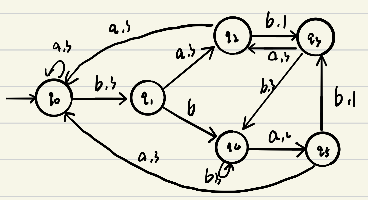展开全文• 形式语言与自动机理论--第三章 有限状态自动机的ppt
• 国科大《自然语言处理》2020春季学期课程学习笔记，第三章形式语言与自动机
国科大《自然语言处理》2020春季学期课程学习笔记，第三章形式语言与自动机。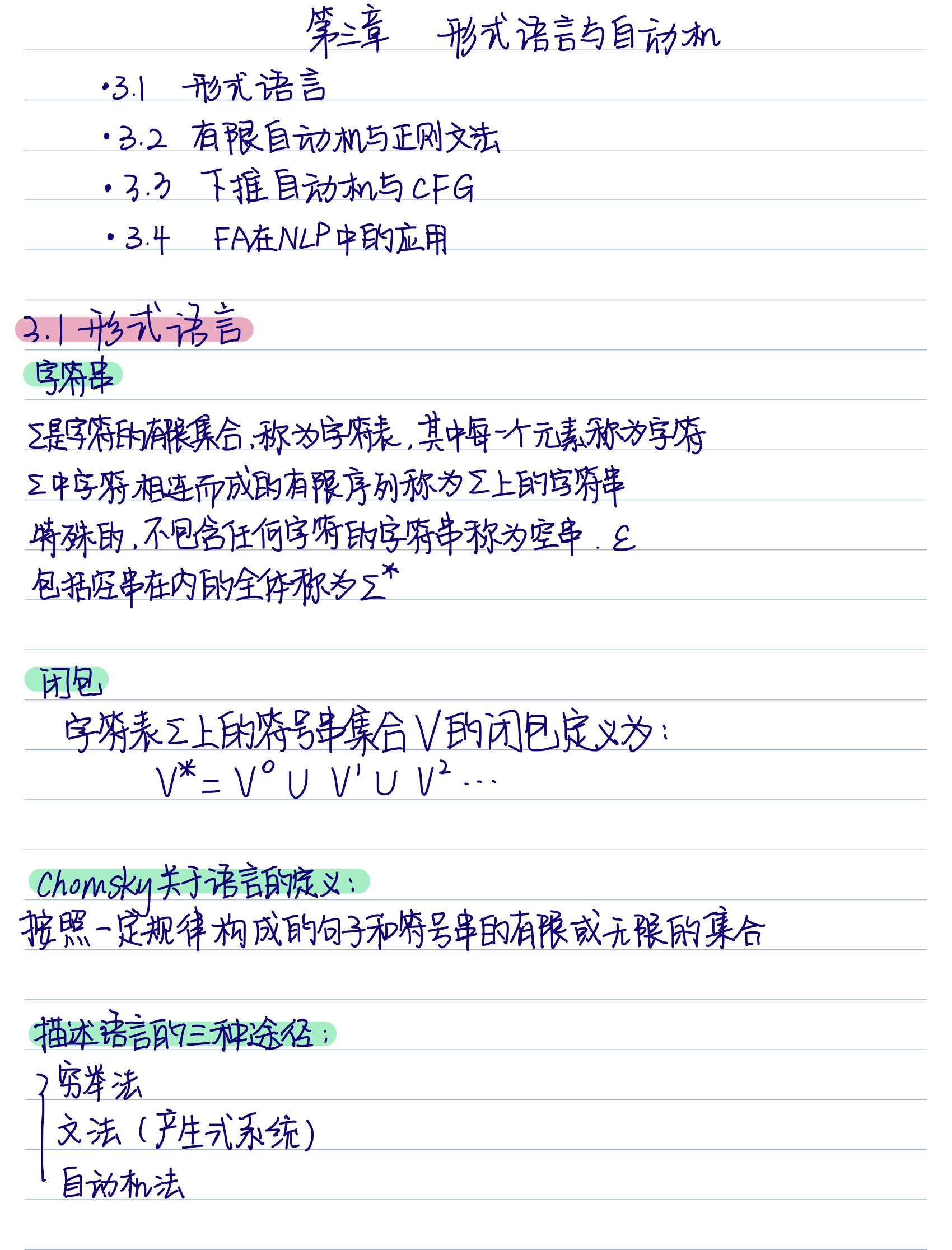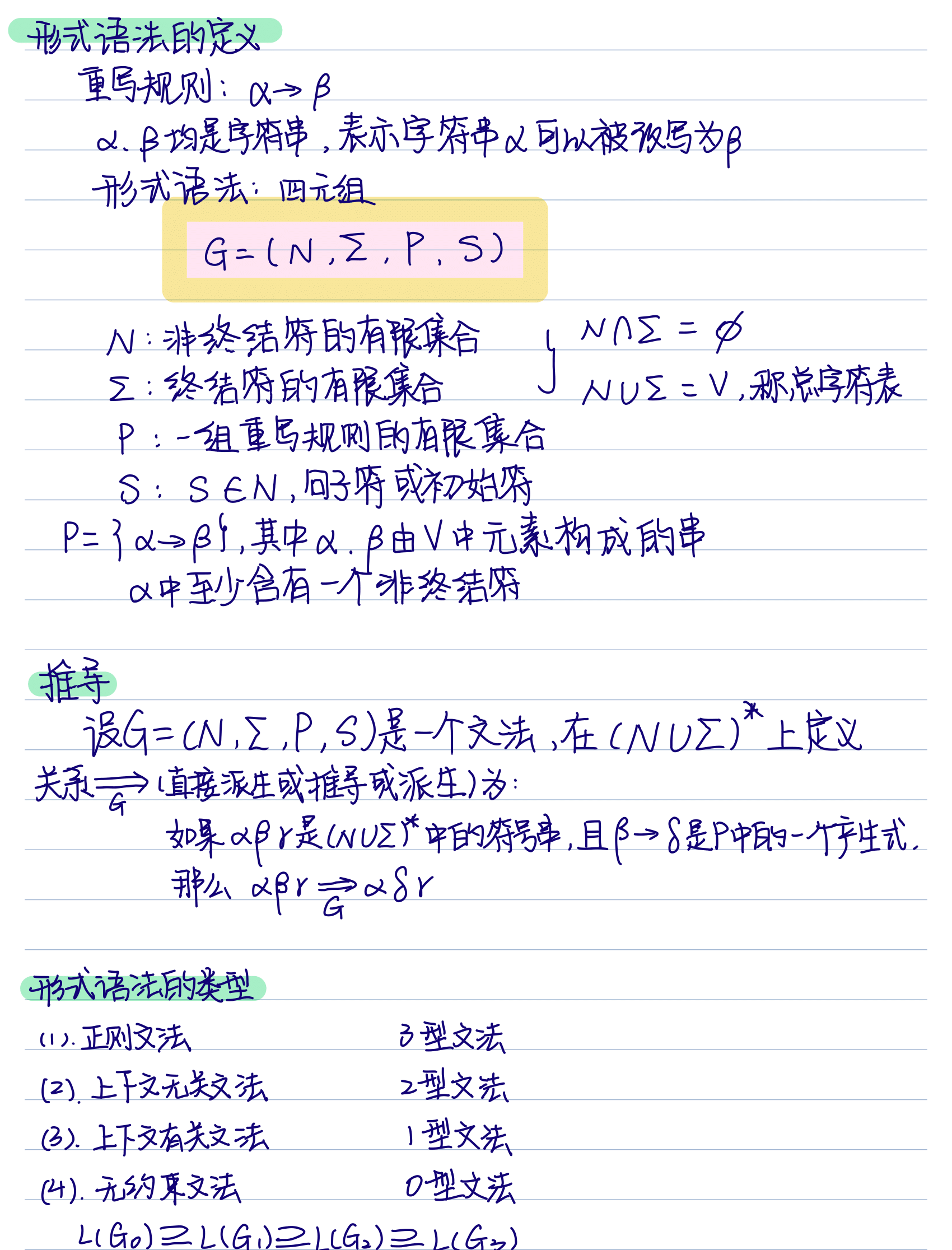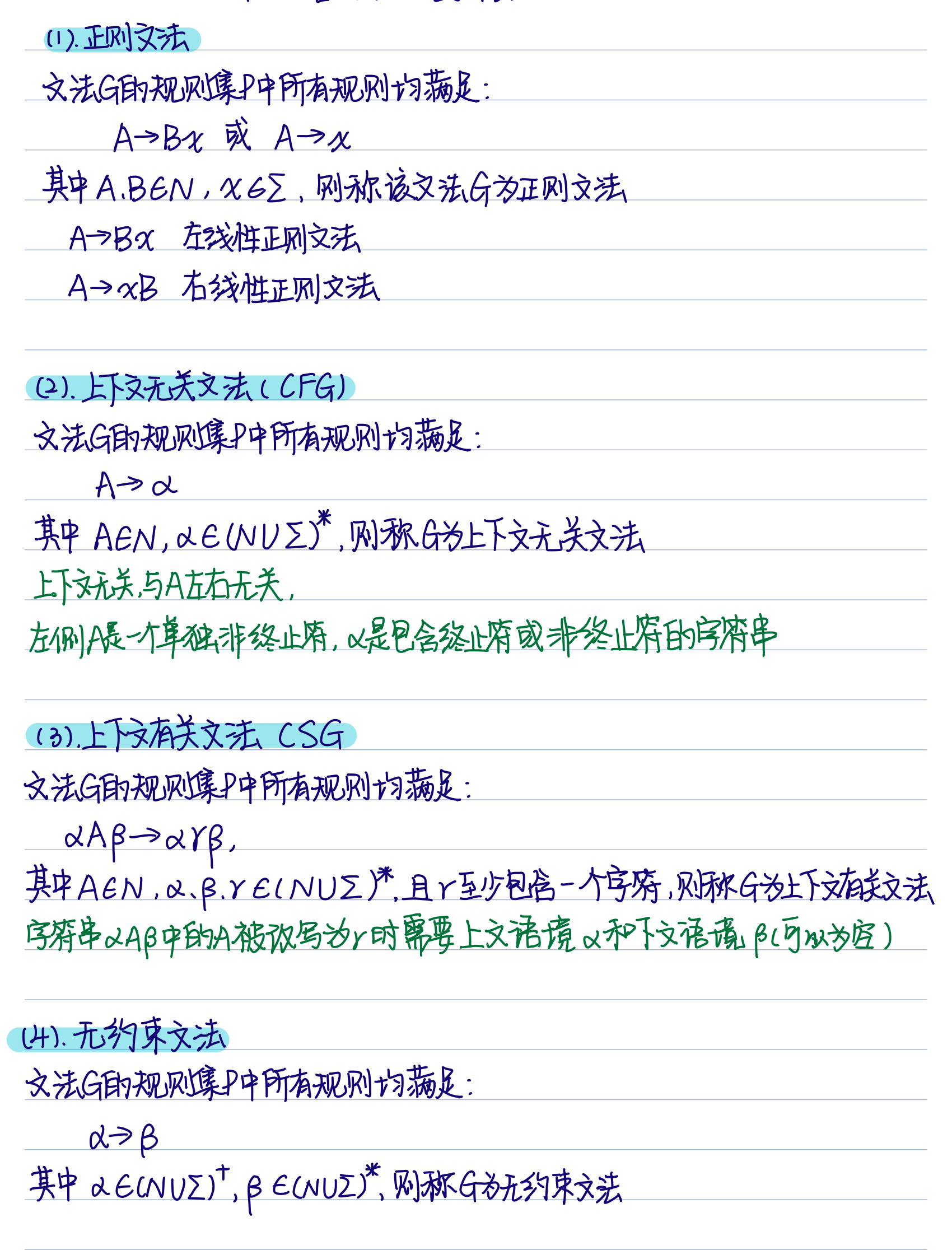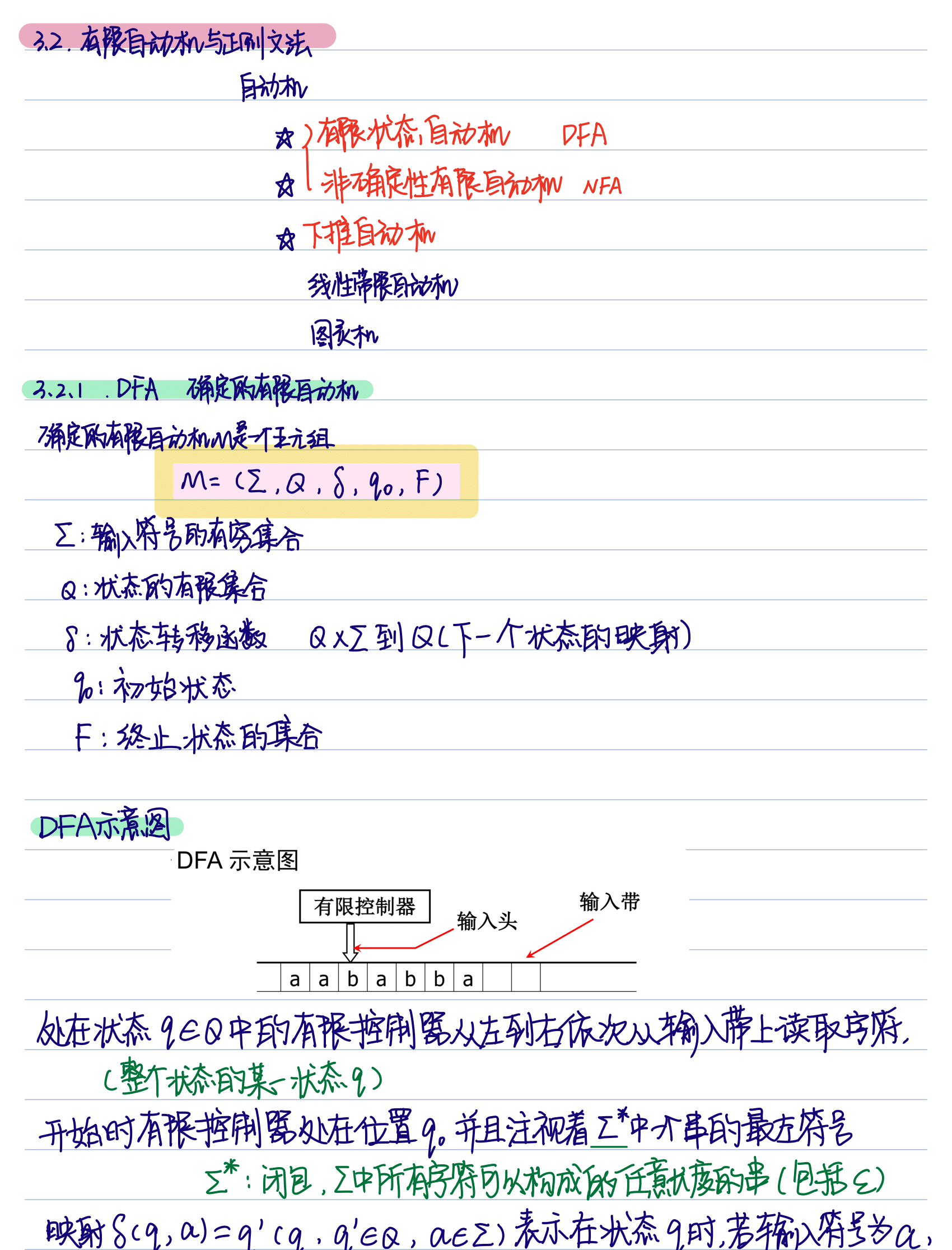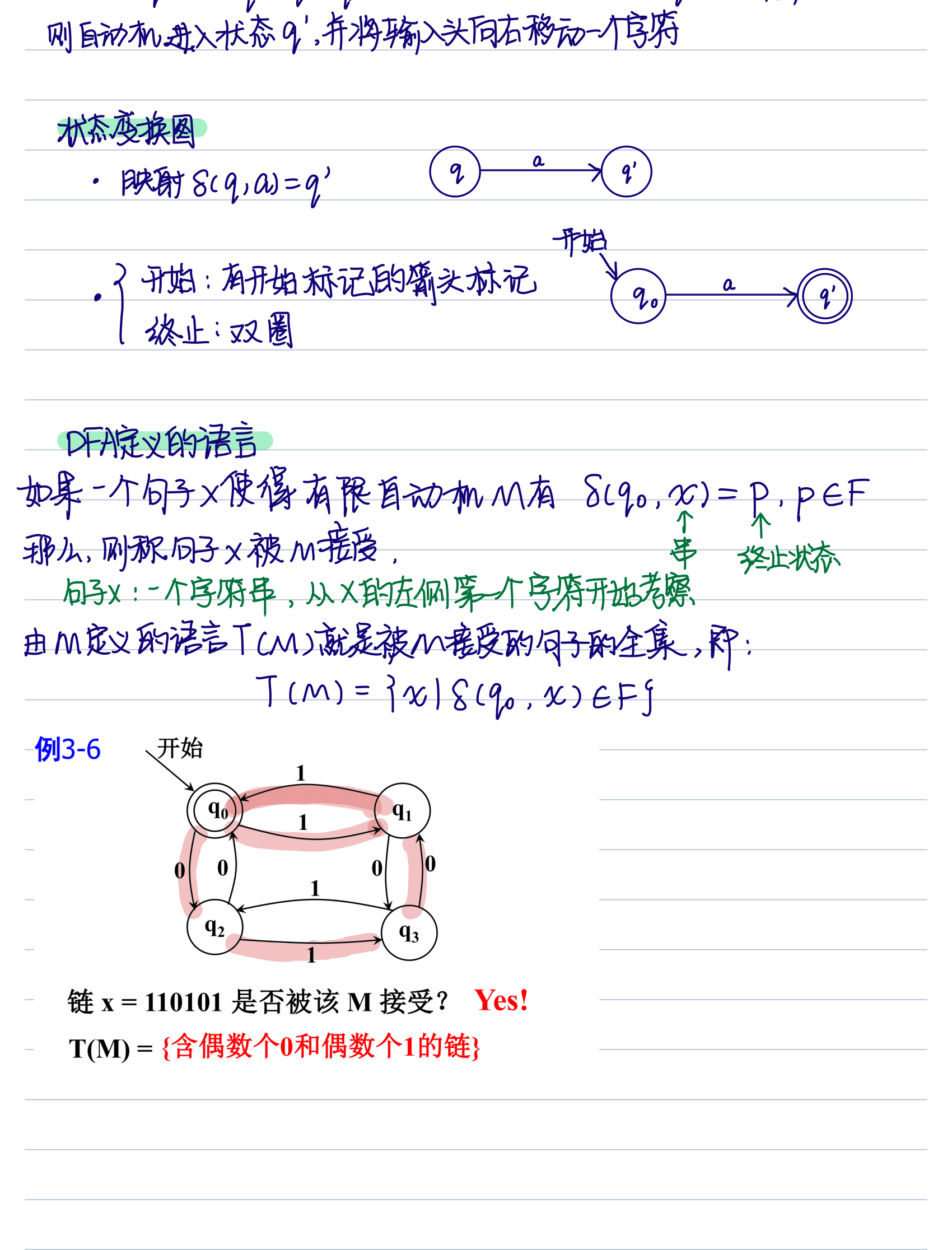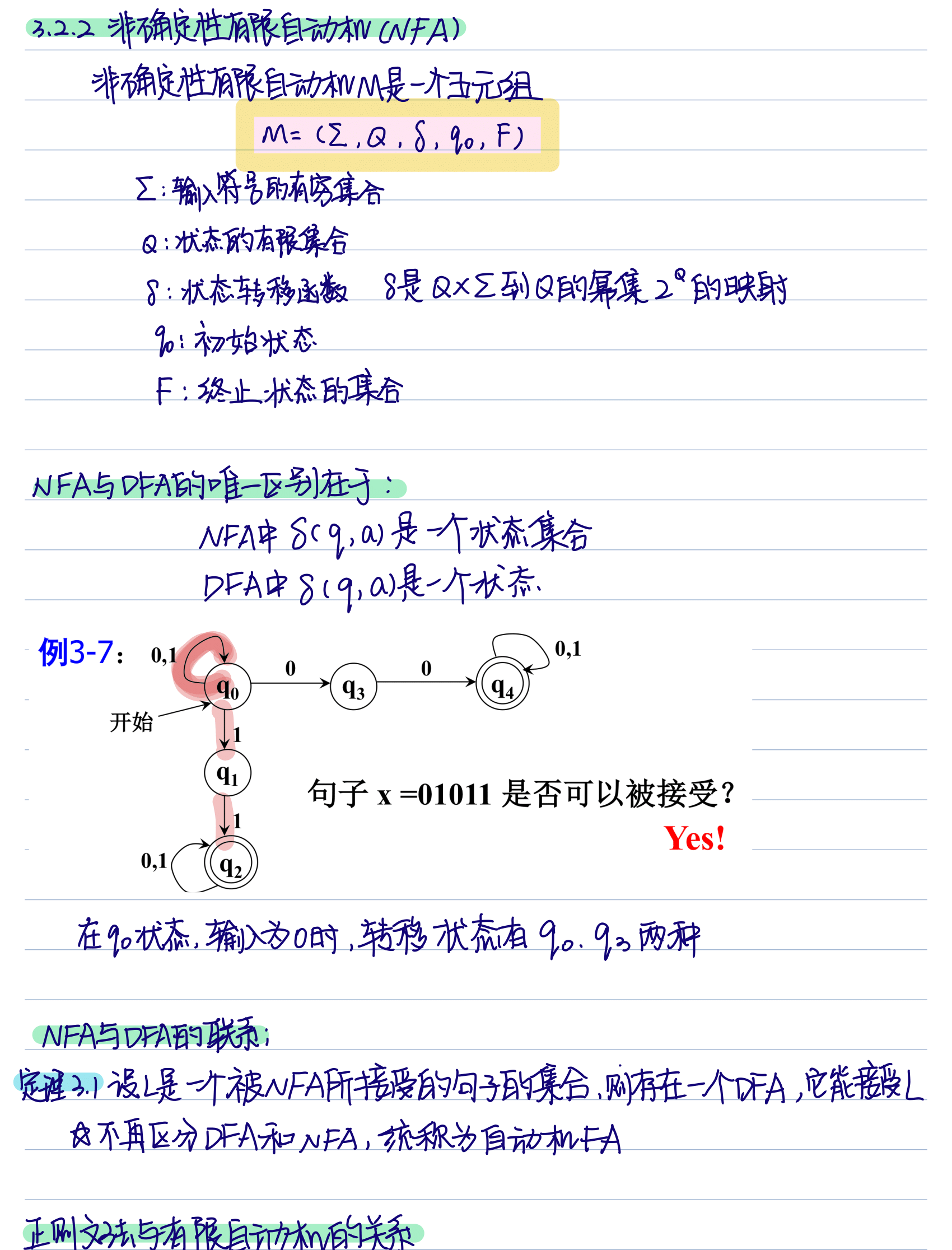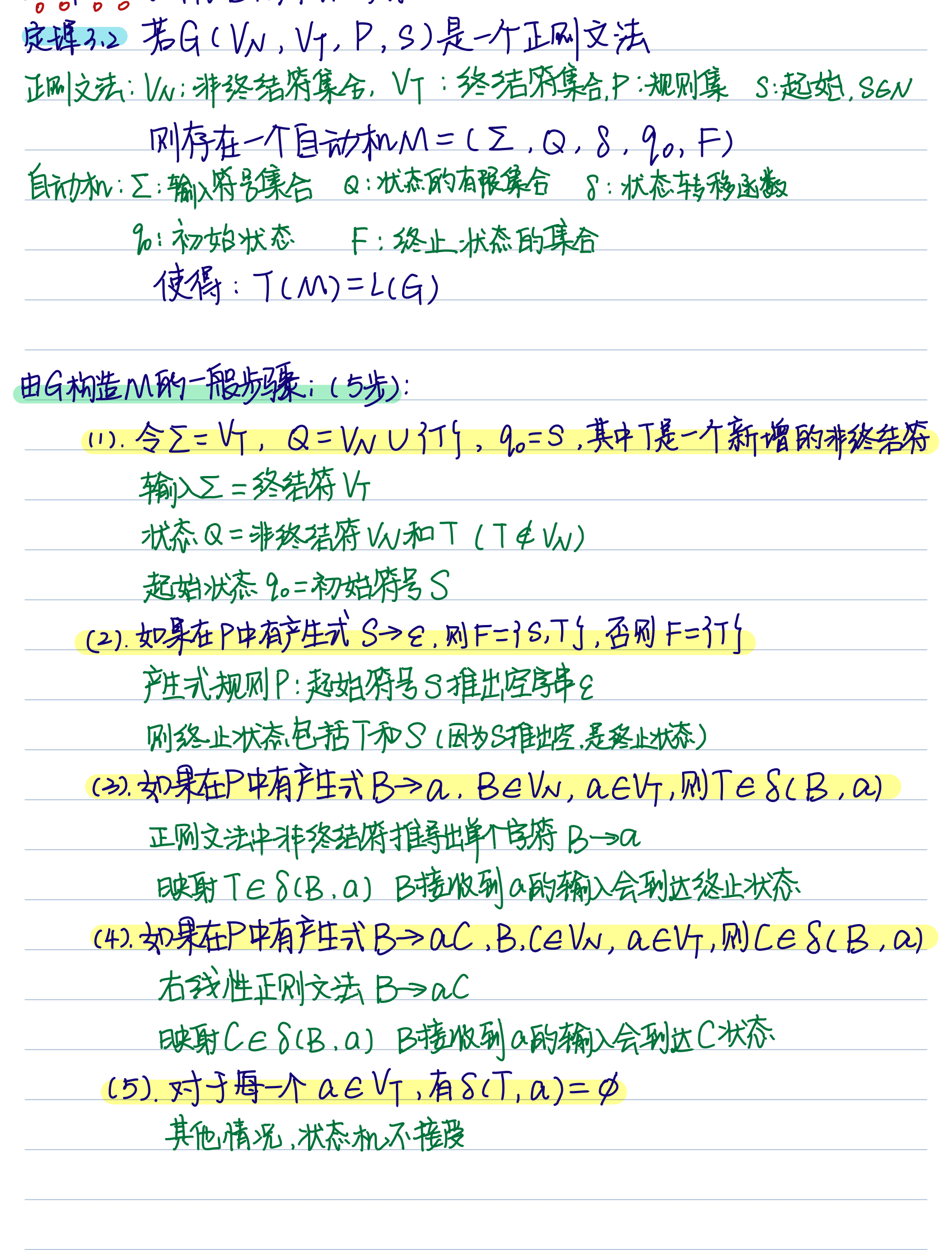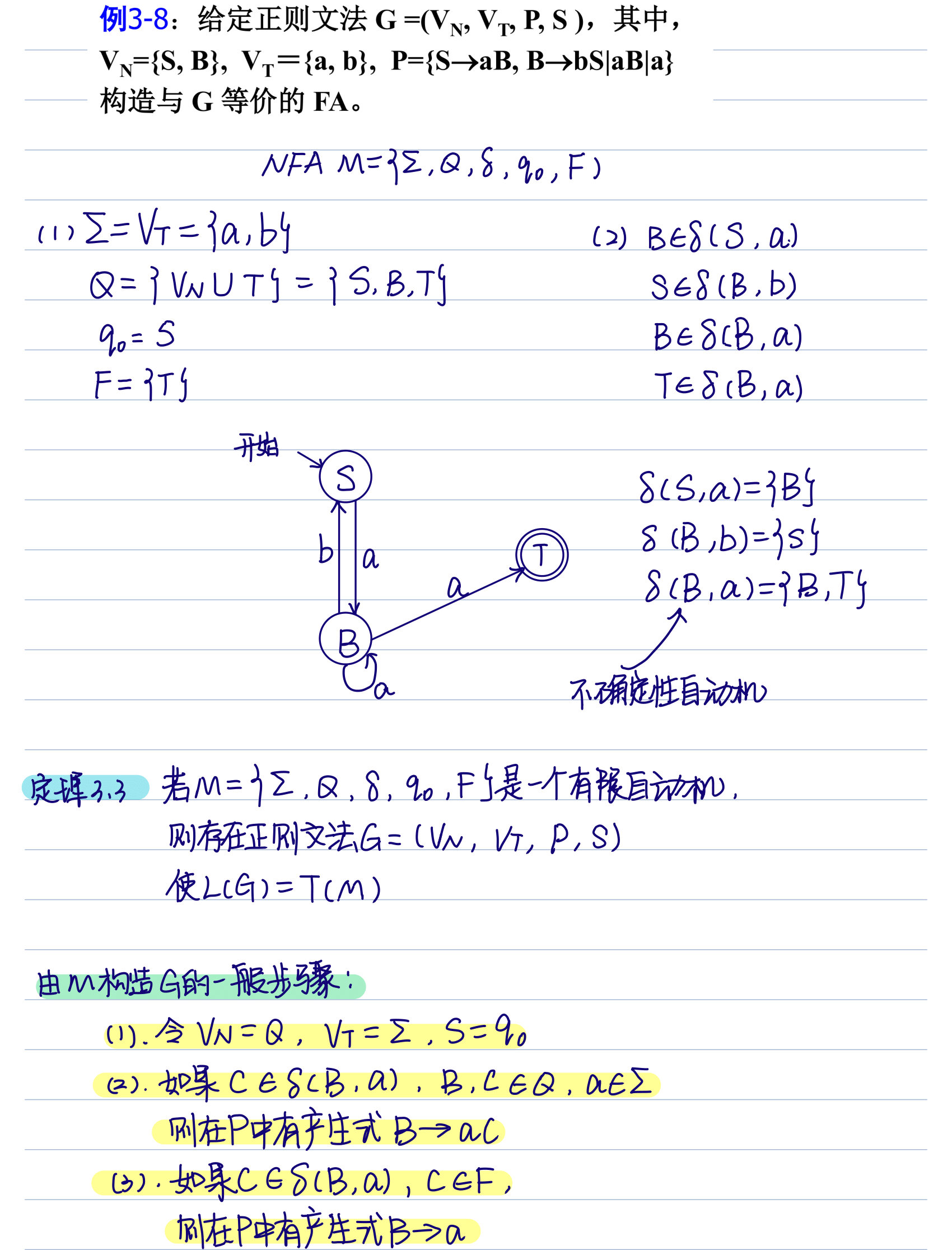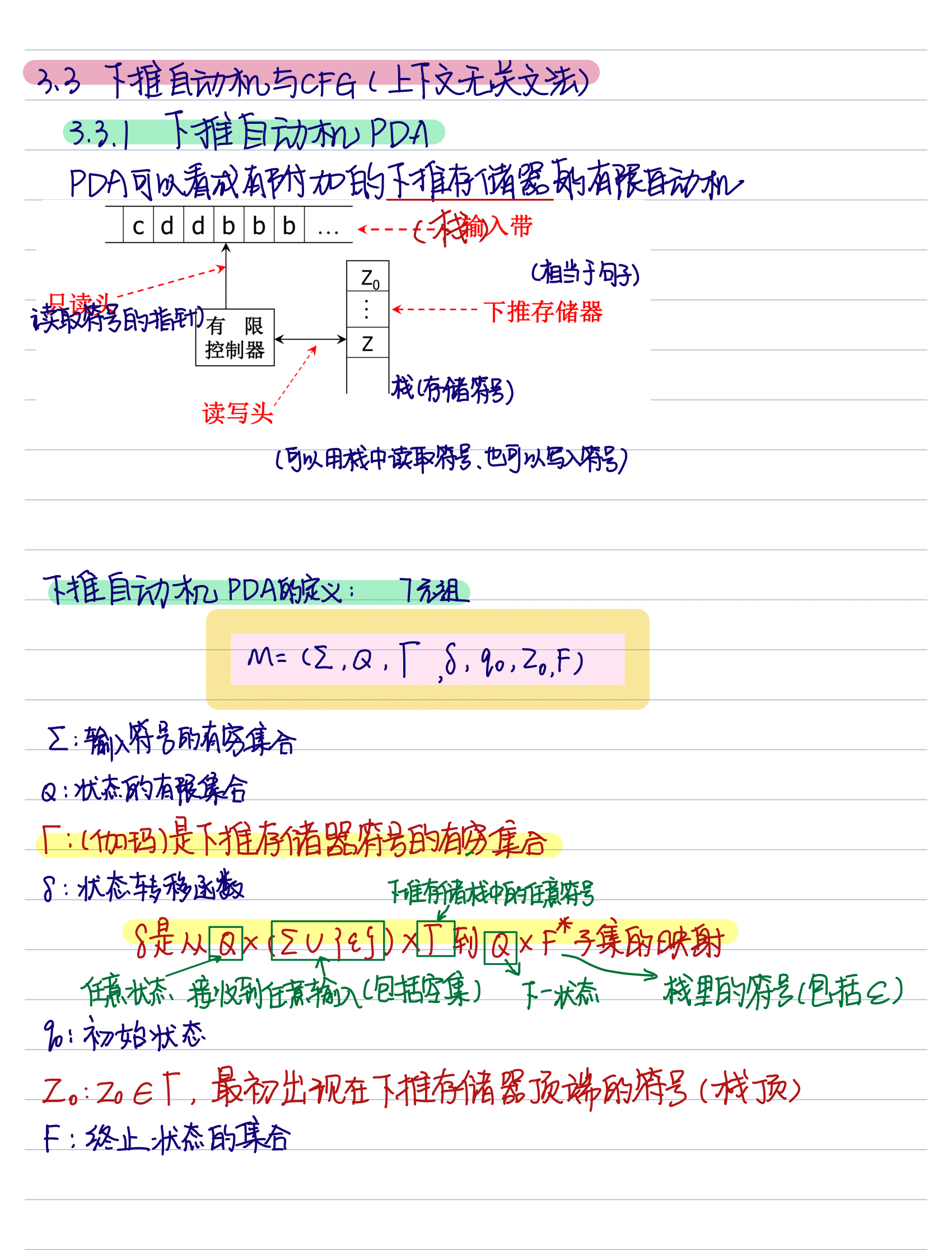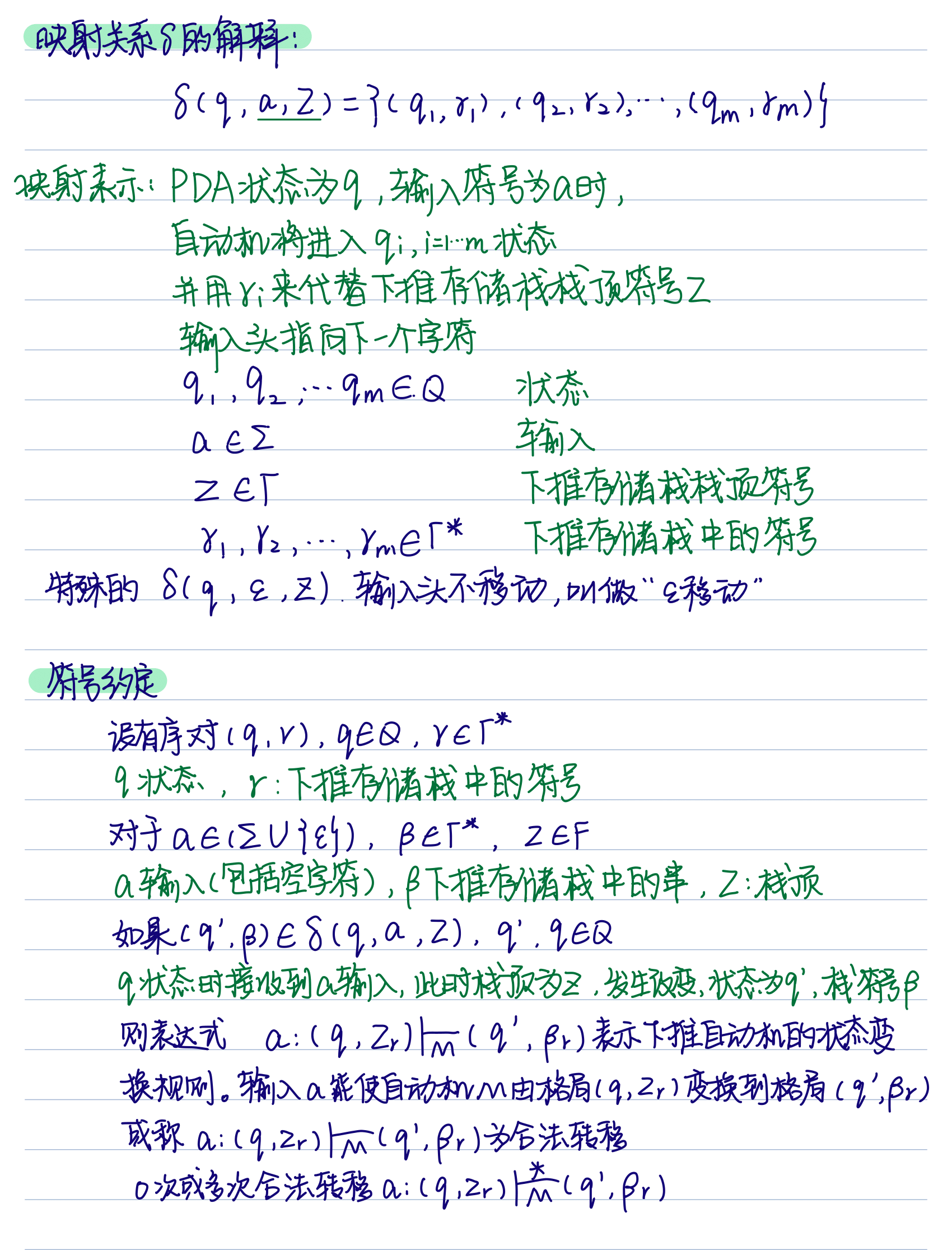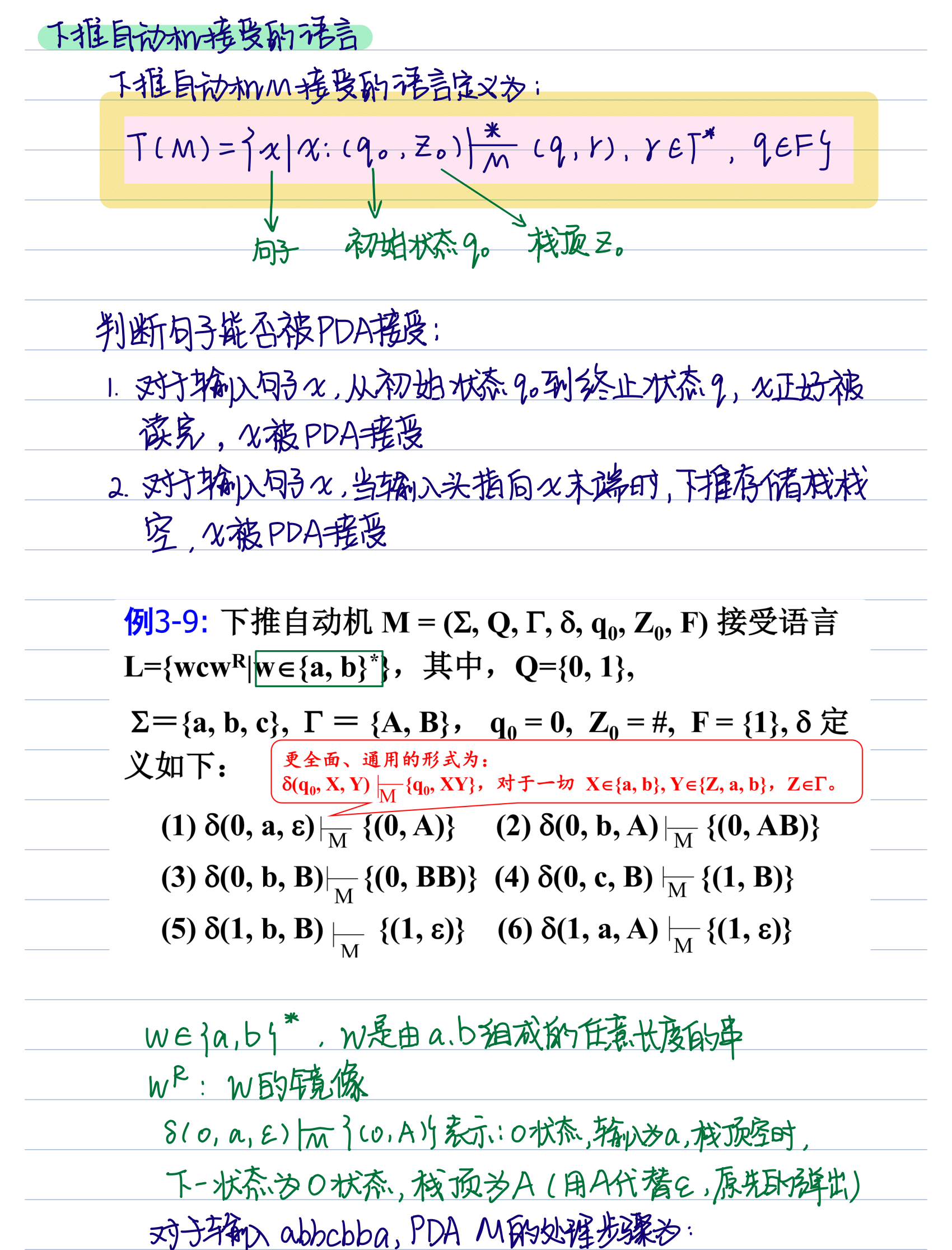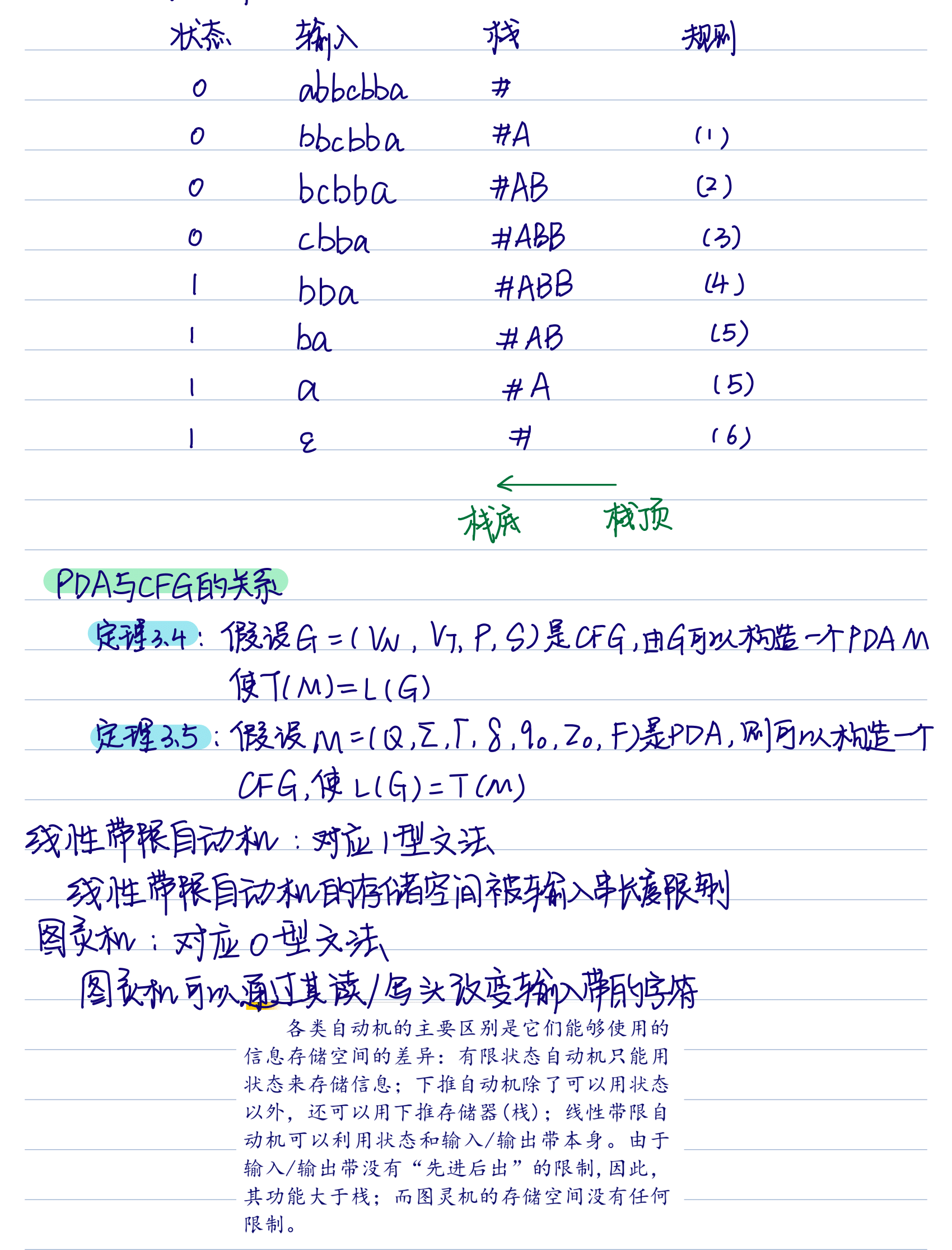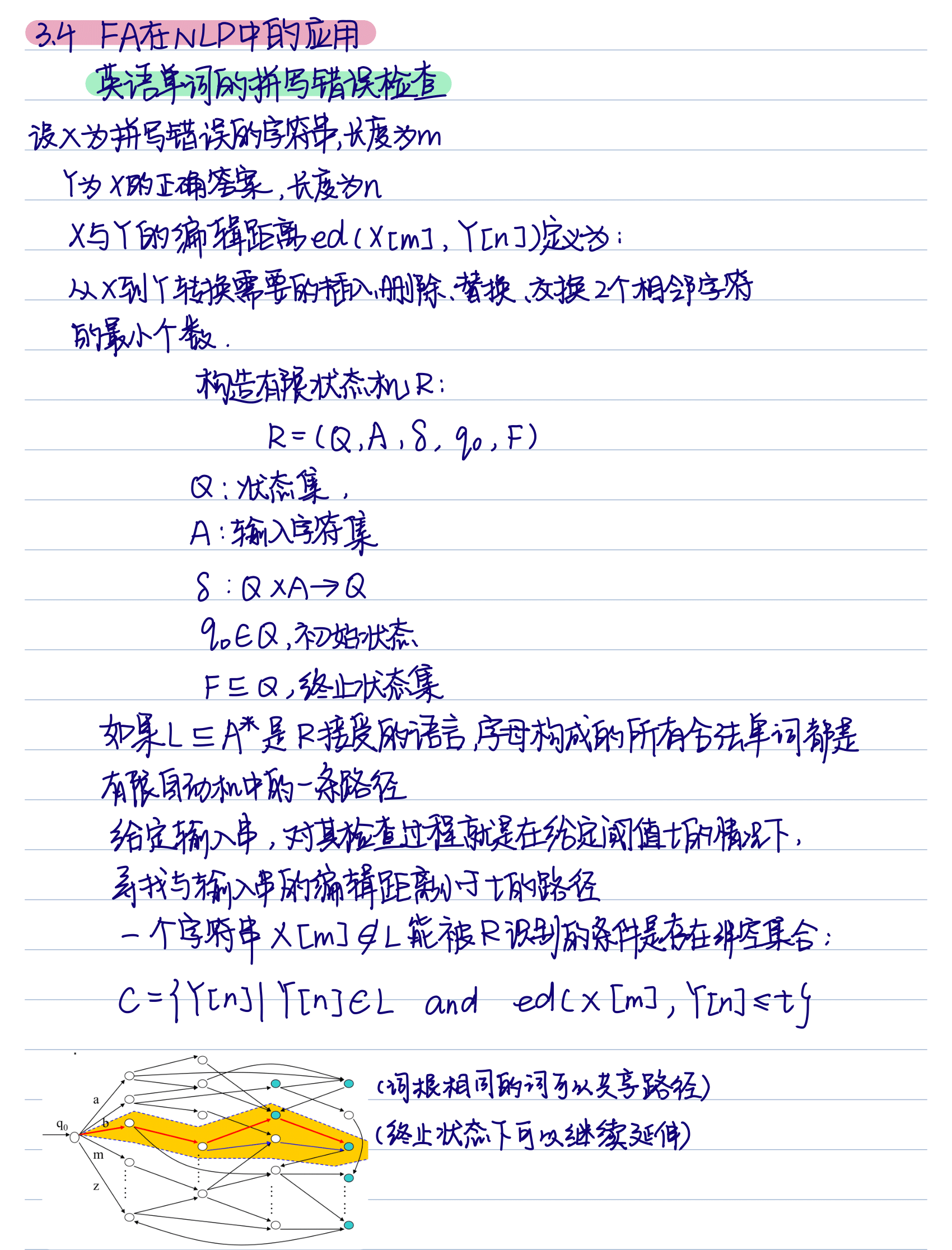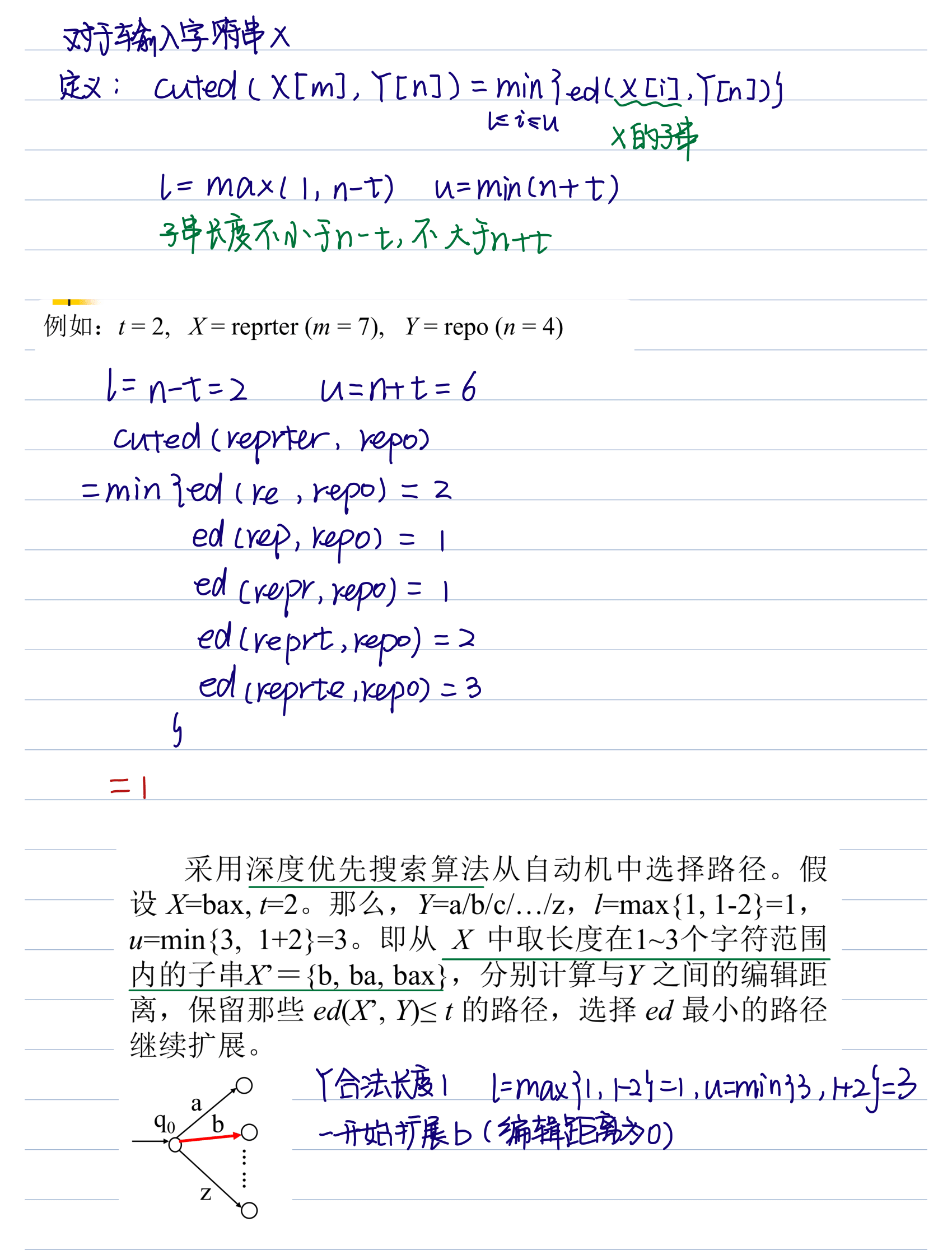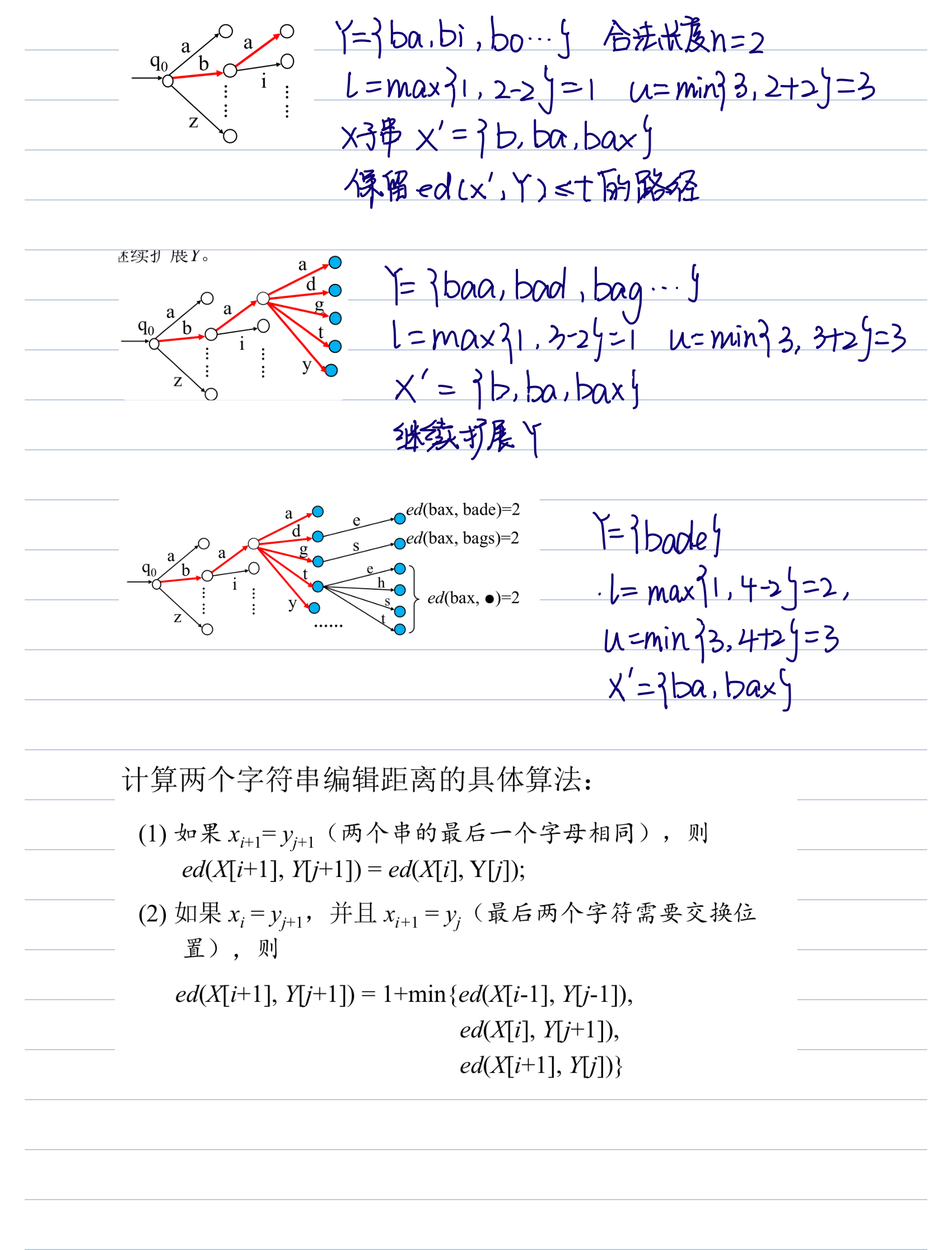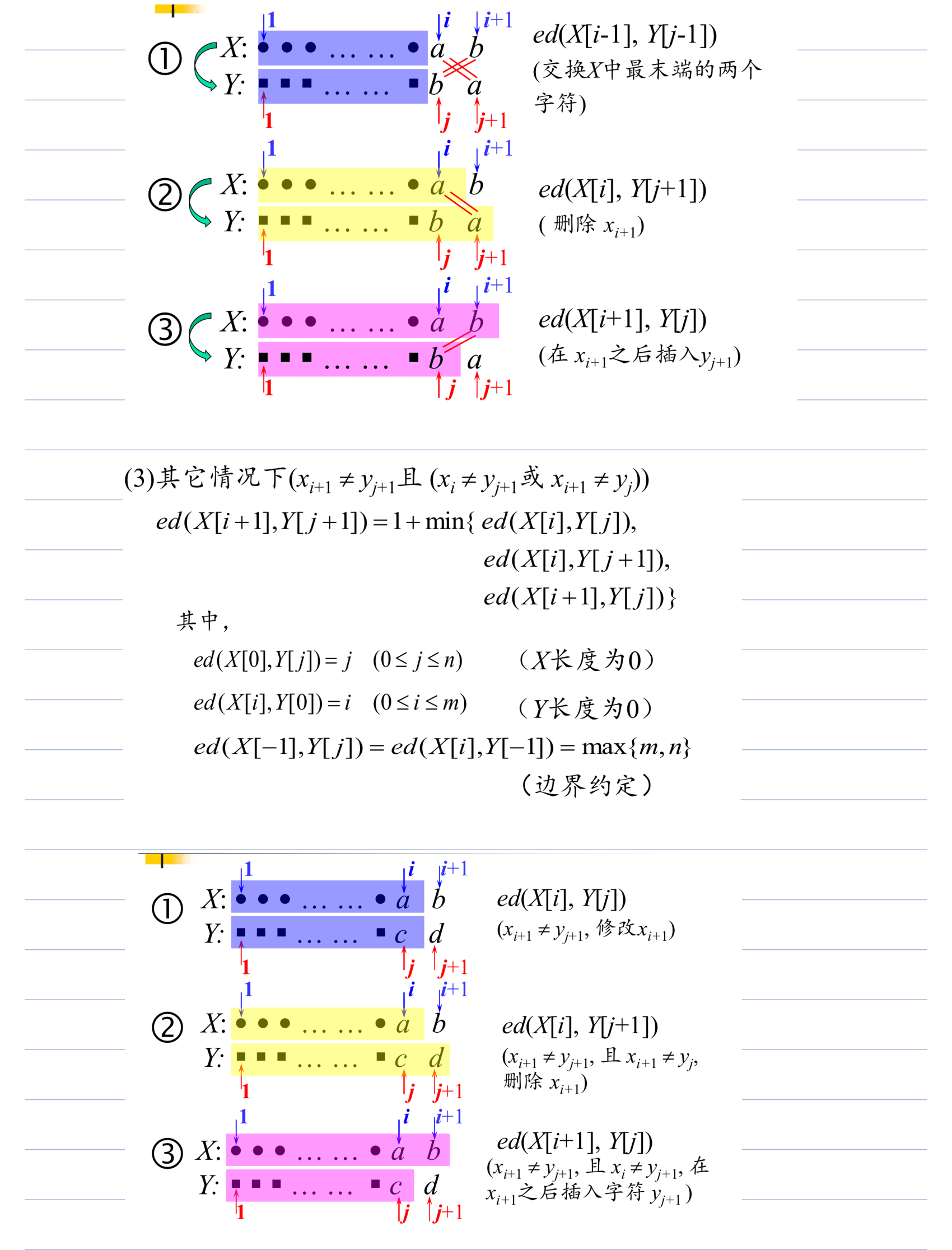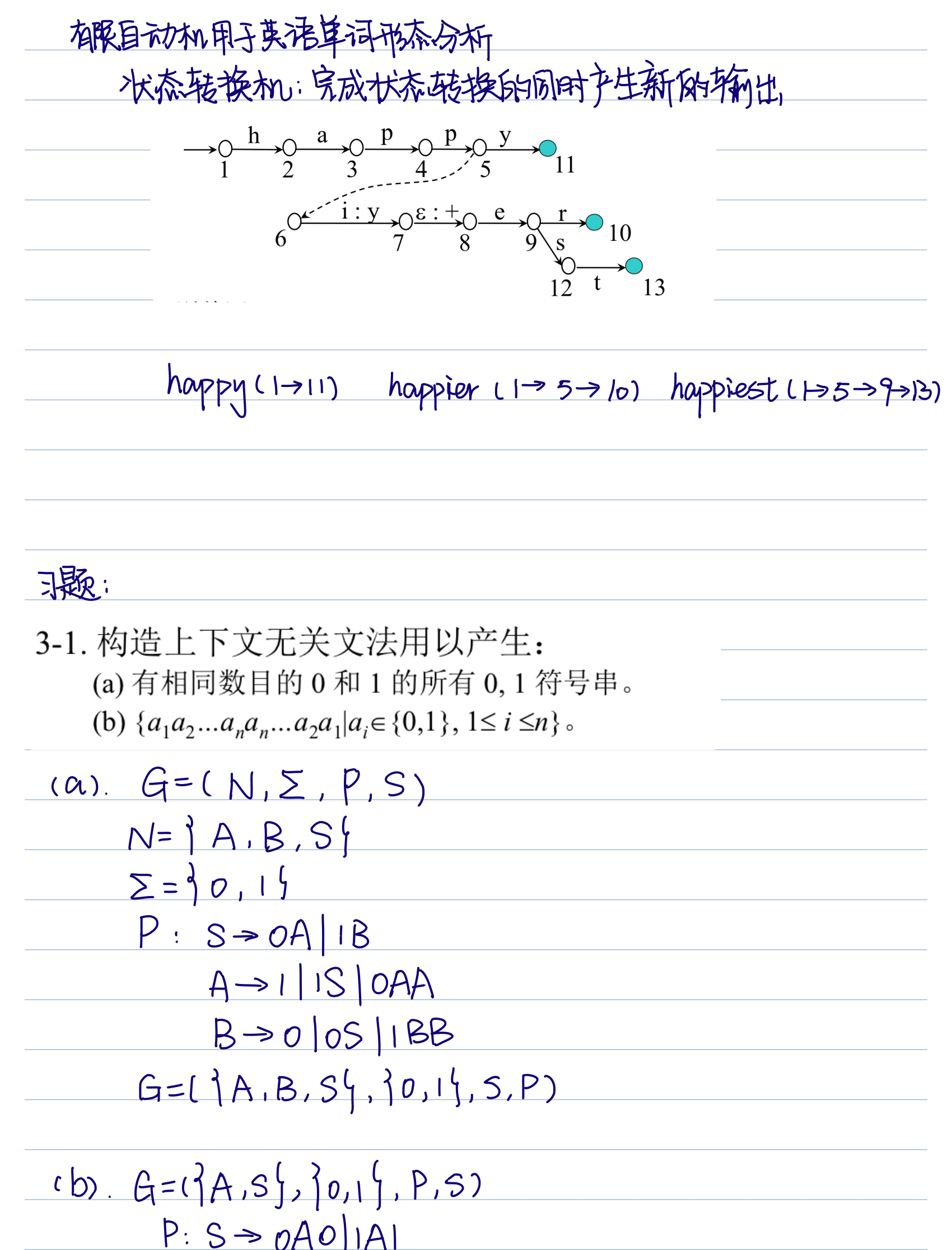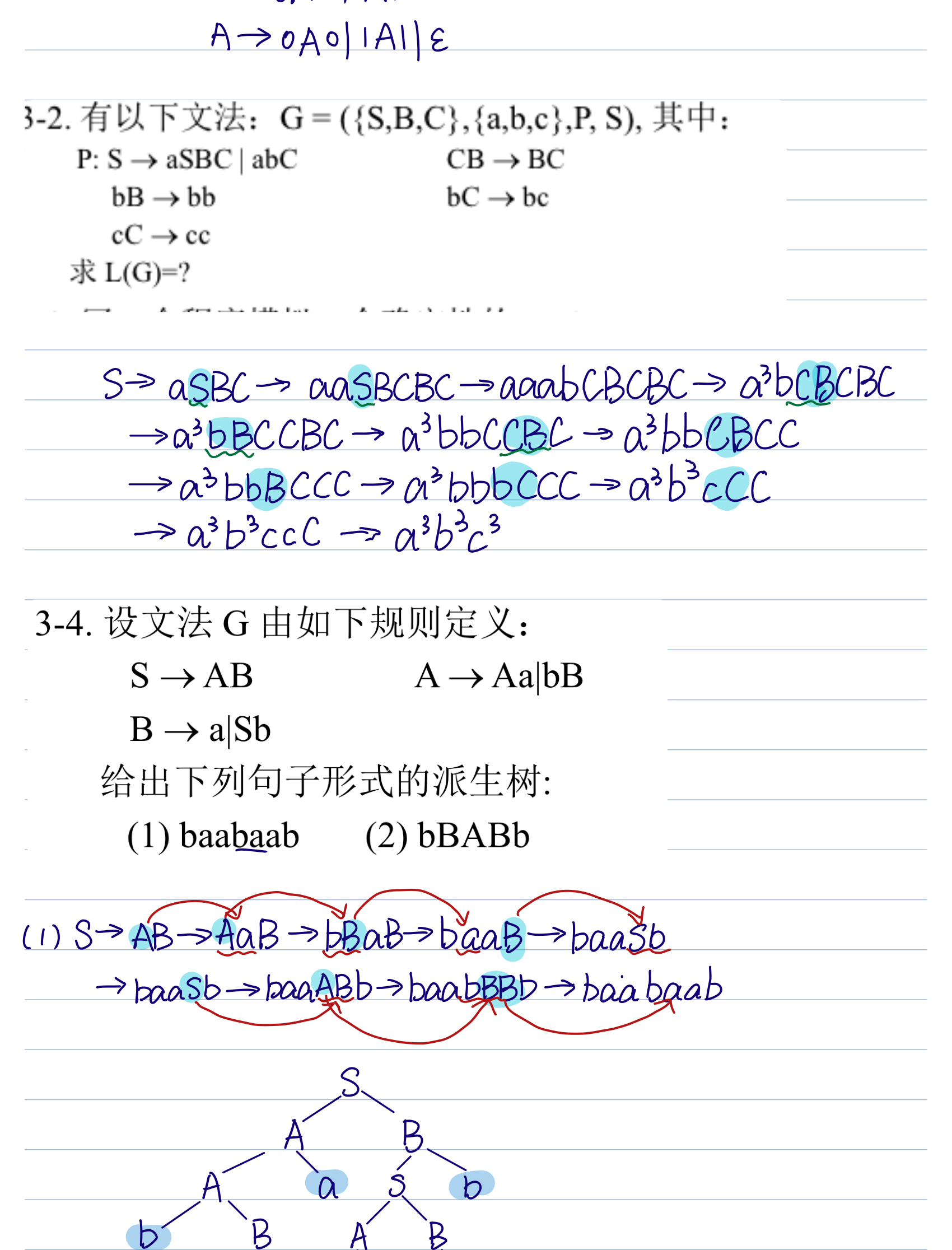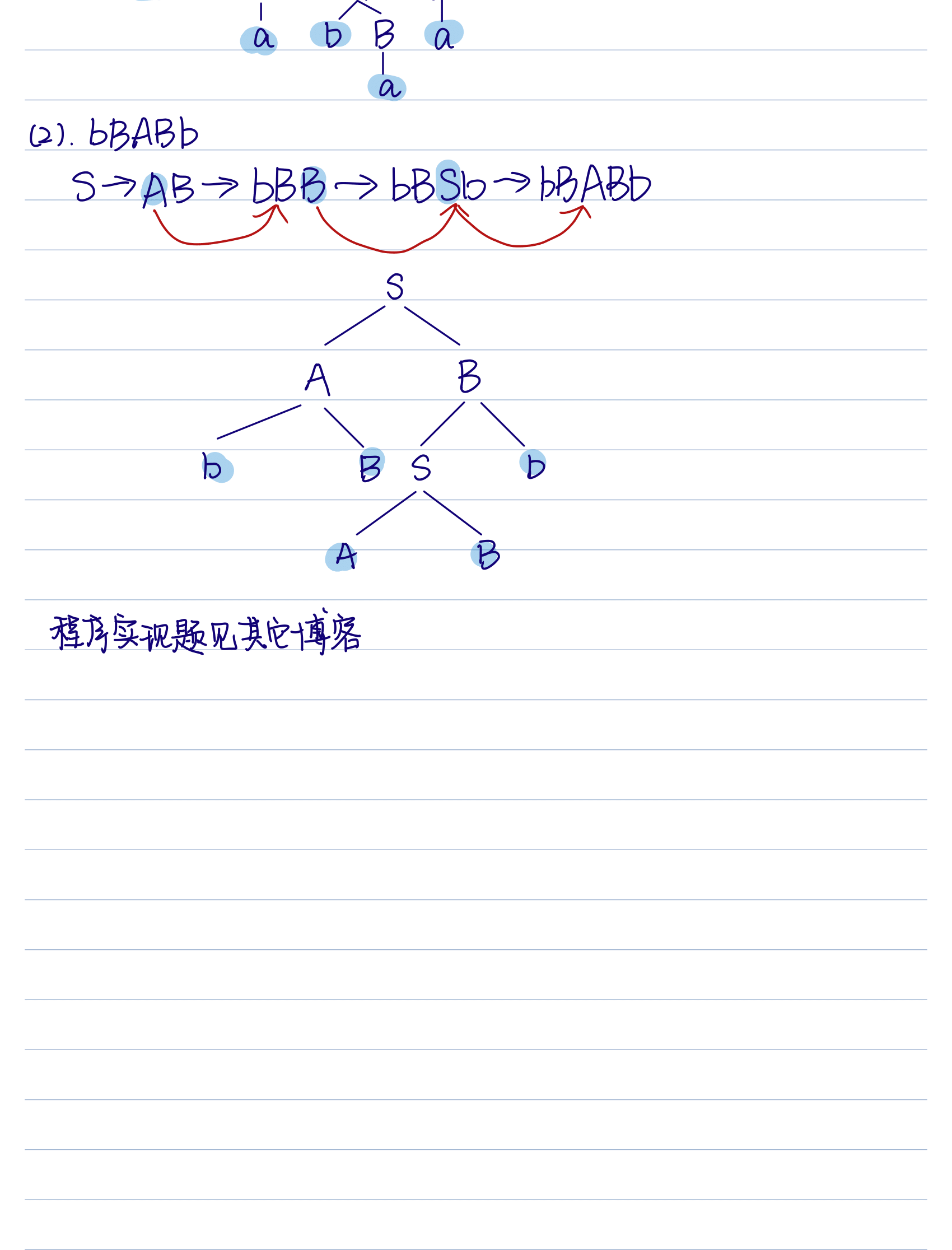展开全文• 新版形式语言与自动机第三章的答案，是北邮版的教材。
• 主要内容： 上下文无关文法（CFG） Chomsky范式和，Greibach范式 确定下推自动机、非确定下推自动机（Push...确定的下推自动机对应于上下文无关语言的一个子集（大部分的程序设计语言）。 3.1 推导树二义性 ...
文章目录3.1 推导树与二义性3.2 上下文无关文法的改写3.2.1 CFG的最简化3.2.2 CFG的变换3.2.2.1 去除可零化的非终结符3.2.2.2 去除单产生式3.2.2.3 去除左递归（递归文法）3.3 chomsky范式、greibach范式3.3.1 改写为chomsky范式3.3.2 改写为greibach范式
该章主要内容：

上下文无关文法（CFG）
Chomsky范式和，Greibach范式
确定下推自动机、非确定下推自动机（Pushdown Automaton）
对任何CFA都能找到一种具有特有形式 的等价CFG（Context-Free Grammar）

上下文无关文法对应的识别器是下推自动机。
确定的下推自动机对应于上下文无关语言的一个子集（大部分的程序设计语言）。
3.1 推导树与二义性
定义：Tree是CFG G=(N,T,P,S)的语法树，是一颗有序树。

树根：S
枝结点是非终结符
叶子结点是终结符或ε
枝特点A有直接子孙ｘ1ｘ2…ｘi,则A→ｘ1ｘ2…ｘi

例：G＝(｛E｝，｛+，＊，i,（，）,｝，P，E)
E→ E+E｜E＊E｜(E)｜i
句子：(a*a+a)
定义：CFG G=(N,T,P,S)如果存在，S ω G是有一棵叶子为ω的语法树
定义：CFG G是二义的 等价于 ω∈L(G),有两棵不同的语法树(叶子为ω)
定义：CFG G是二义的 等价于 ω∈L(G)，有两T不同的最             左(右)推导
文法二义无法推出语言二义，语言二义可以推出其文法均二义
文法的二义性的举例：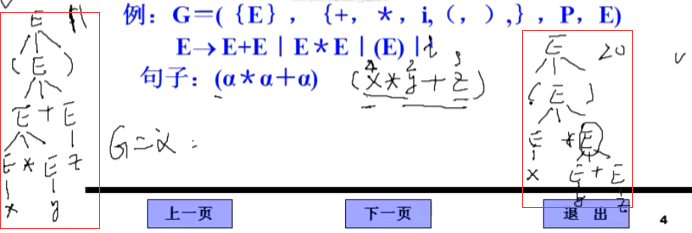左边的树在展开的时候先展开运算符，右边的树在展开的时候先展开+运算符，如果运算的定义无结合律则会存在二义性。23+5=11；2*(3+5)=16
3.2 上下文无关文法的改写
我们约定俗成：大写为非终结符，小写为终结符
为了解决文法可能存在的二义性问题，我们引入上下文无关文法的改写。在不改变文法描述能力前提下改写文法满足一定要求。改写目标：将CFG改写成某种标准形式.
(1) 改写成Chomsky范式：            A→ BC｜a   A,B,C∈N a∈T
(2) Greibach范式            A→ a阿尔法    阿尔法∈N*,  a∈T
3.2.1 CFG的最简化
但是在改写之前我们必须要做一件事，就是CFG的最简化
我们要分别有用符号和无用符号。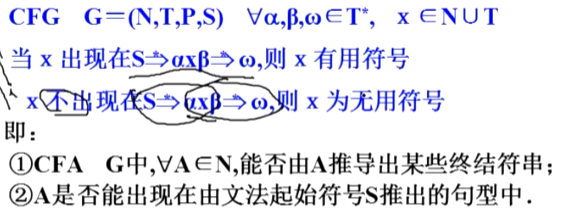补充：START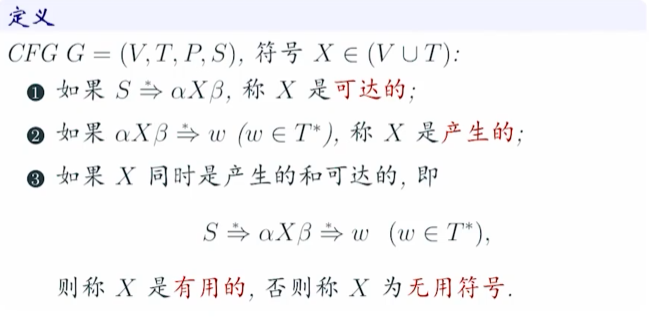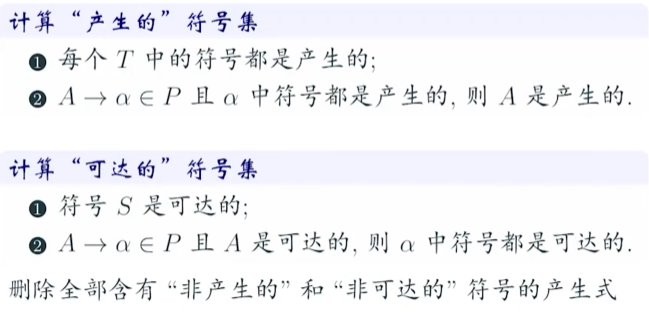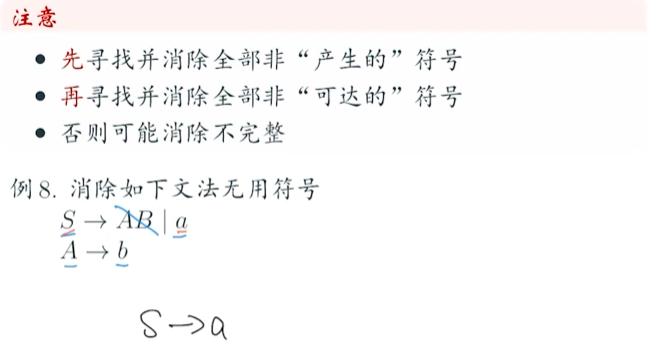END
4月29日 录播 1:10:54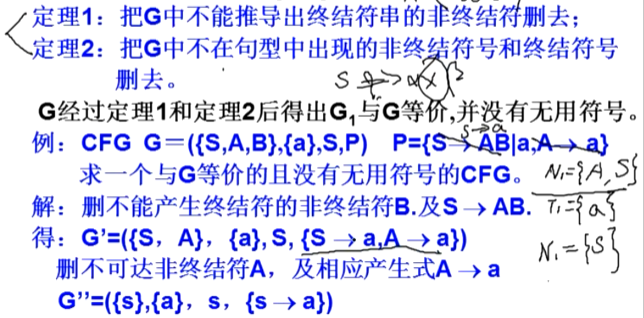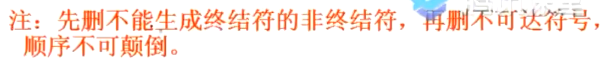3.2.2 CFG的变换
到这里我们来总结一下上面的内容。我们的目标是去除文法的二义性，首先要去除无用符号，接下来要去除可零化的终结符。我们这节就来说“去除可零化的非终结符”。
3.2.2.1 去除可零化的非终结符
定义:若在CFG中，一个非终结符经过若干次推导可以推出空字，那么这个非终结符称为可零化的非终结符。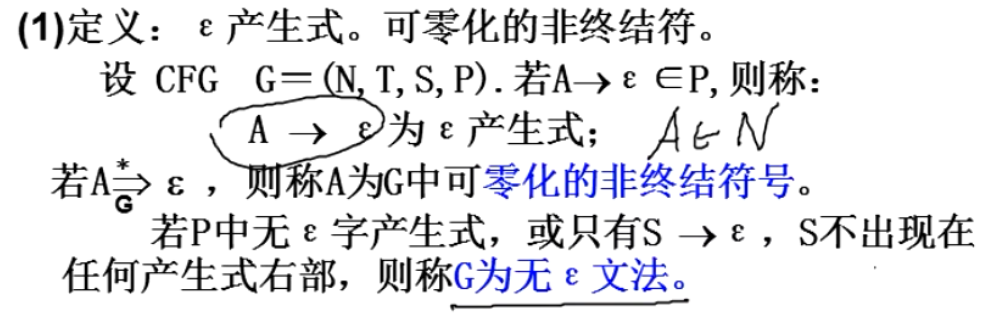举例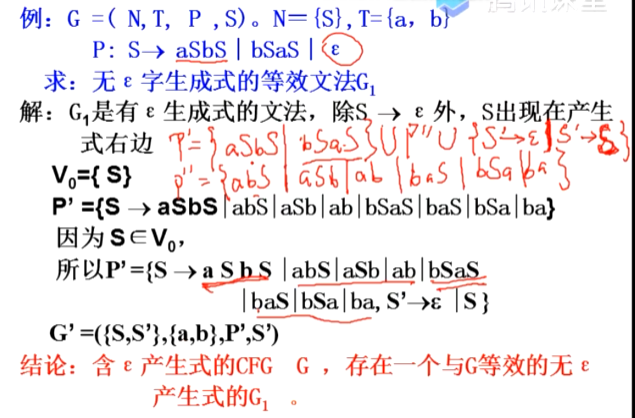从P中去掉 单独非终结符直接产生单独空字 的产生式，去掉后的产生式集记为P’
把第一步中去掉的产生式代入其他产生式，把新的产生式加入P’’
把P’’ 并入 P’，再加入新的非终结符，假如为S’，再把S’推出S和S’推出空字加入P’

则最终结果是G’ = ({S, S’}, {a,b},P’,S’)
具体推导过程参加腾讯课堂录播“文法改写”第1:35:00
补充：这个办法有点生搬硬套了，一般方法参见https://www.bilibili.com/video/BV1oE4116794?p=24，9分04秒。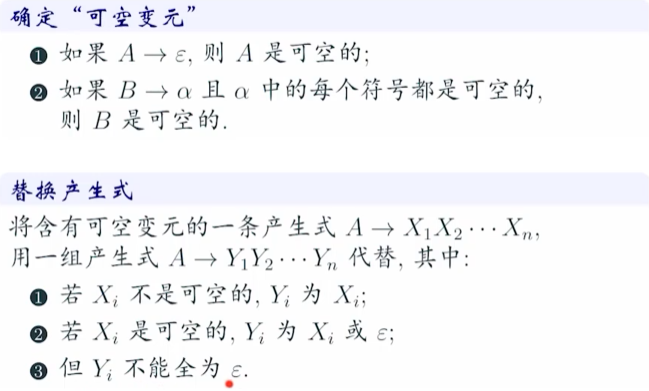3.2.2.2 去除单产生式
单产生式形如 “A → B”，且AB均属于N。
当G是无空字产生式的CFG，但存在单产生式，可以利用算法2构成一个无单产生式的等效方法G1。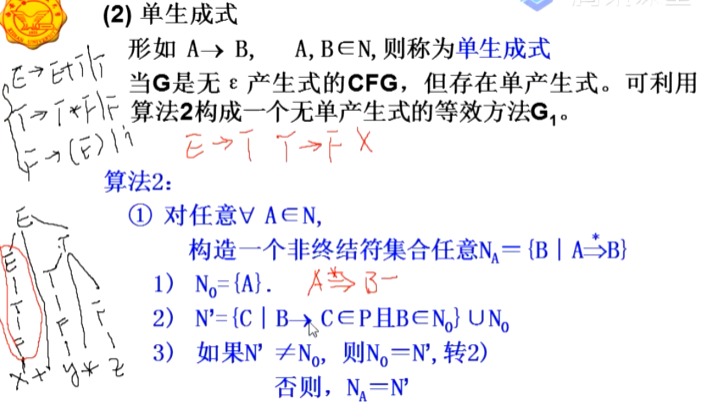例子：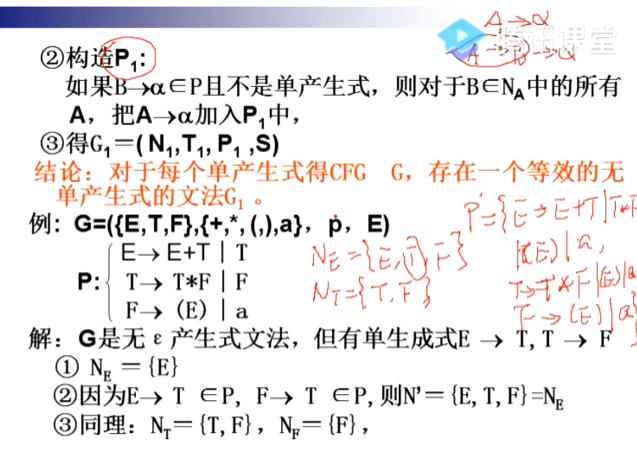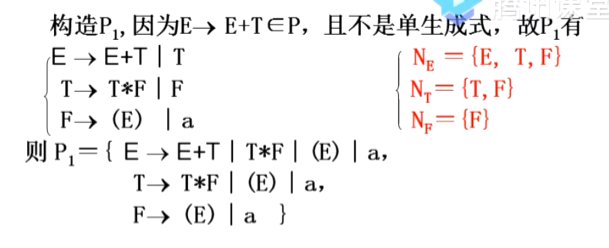视频“文法改造2:03:00”，
补充：参照https://www.bilibili.com/video/BV1oE4116794?p=24，9分04秒
3.2.2.3 去除左递归（递归文法）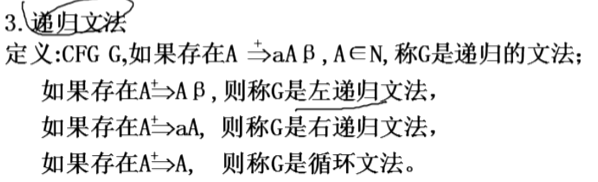3.3 chomsky范式、greibach范式
我们先来总结一下上面的内容，从头到尾其实都在说CFG的改写。分别是：

去无用符
去空字
去单产生式
去左递归，这里还可以细分为直接递归和间接递归
去二义性

而我们今天要继续讲把CFG转化为C范式或G范式。
3.3.1 改写为chomsky范式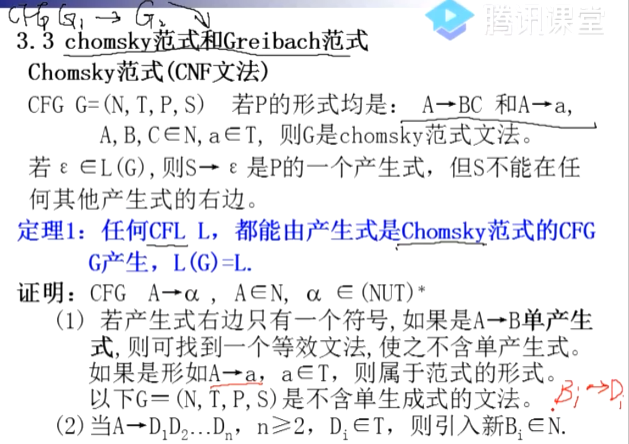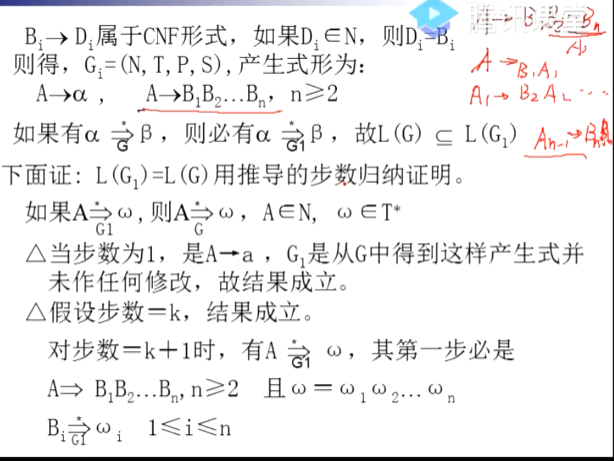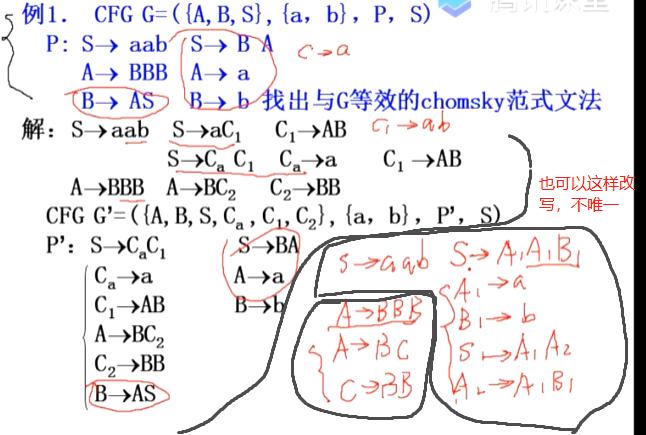补充：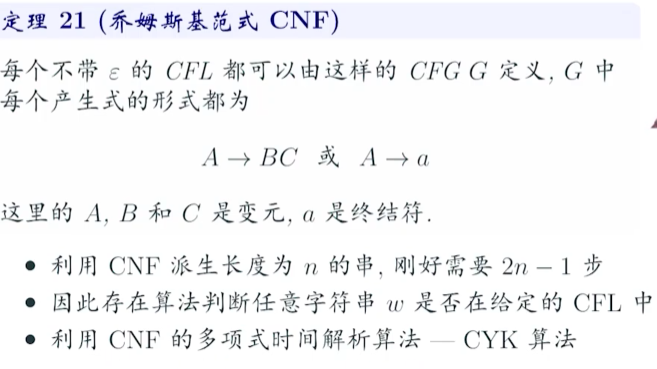3.3.2 改写为greibach范式
定义：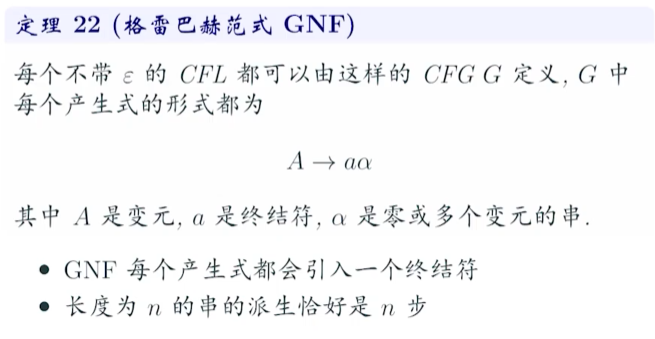由于在改写为G范式之前还要再消一次左递归，详情见视频G范式34min46s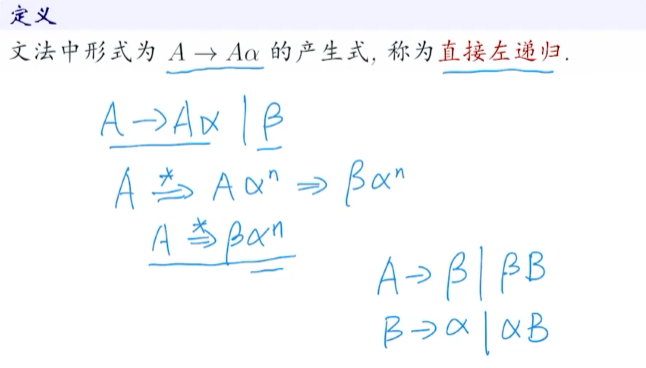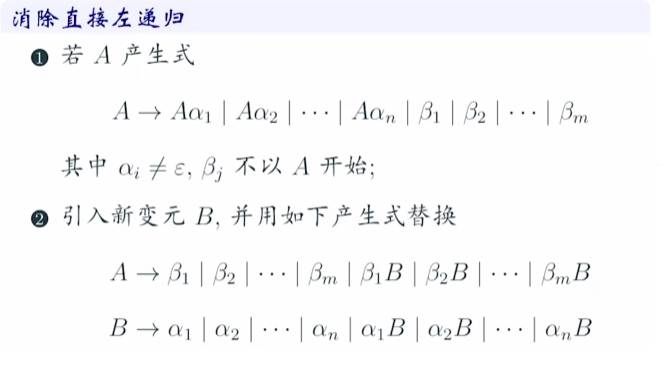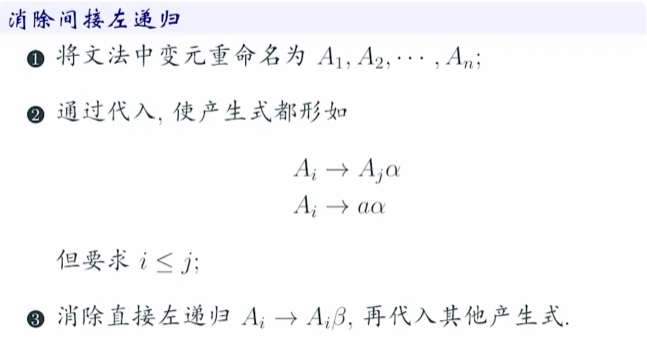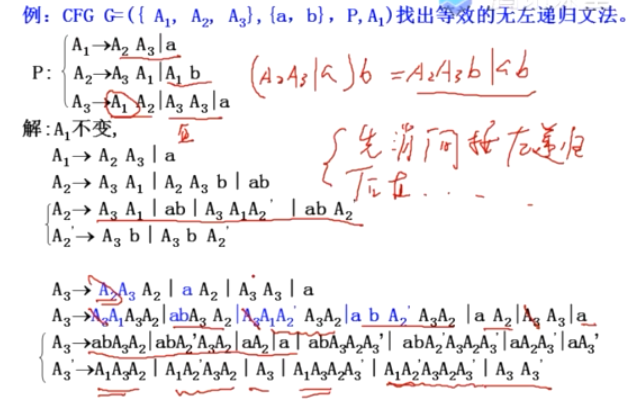正文
方法一：直接转法
从CFG直接转成G范式
参见https://www.bilibili.com/video/BV1oE4116794?p=25，视频最后一个例题。
方法一：两步转法
我们先从CFG改写为C范式，再有C范式改写为G范式。


展开全文• 我们来回顾一下文法与自动机的区别： 文法：对语言的有穷说明 识别器：有穷的说明无穷语言的一种方法，最简单的识别器就是有穷自动机 文法可以建模，但是文法不能有效地编程实现，因此引出了有穷自动机。 有穷...
• 第三章 图灵机（更高级的形式化手法） 一、 语言及文法 文法系统——一类生成系统 1.1 文法的形式概念 字母表： 字符的有限集合——字母表T 例T={a…z, A…Z, 0…9, +, -, …} 字母表的成员不一定必须是单个的字.....
• 形式语言与自动机理论清华大学蒋宗礼-第三章参考答案
• 1.形式语言 语言描述的种途径： 穷举法——只适合句子数目有限的语言； 语法描述——生成语言中合格的句子； 自动机——对输入的句子进行检验，区别哪些是语言中的句子，哪些不是语言中的句子。 直观意义： 用来...nlp
• 词法分析有穷自动机1、词法分析程序的功能2、正规集、正规式、正规文法、确定的有穷自动机、不确定的有穷自动机的定义。3、正规文法、有穷自动机、正规式者之间的互相转换方法。不确定有穷自动机到确定自动机的...
• 第三章：正则表达式正则语言 正则表达式能定义所有并且只有正则语言。 3.1正则表达式 正则表达式恰好定义了各种形式自动机所描述的相同的语言：正则语言。但正则表达式提供了自动机所没有的东西：一种表达要...
• 学习《统计自然语言处理-宗成庆》这本书时，对理论部分第三章-形式语言与自动机存在许多困惑，因为抽象的概念比较多，而且例子比较少，理解起来比较晦涩，故自己整理下这方面知识，以期巩固知识，加深理解。...
• <br>目录 1、1字符串、字母表和语言 预备知识 1、2图和树 1、3归纳证明 1、4集合 1、5关系 1、6本书提要 2、1有穷状态系统 有穷自动机和正规表达式 2、2基本定义...
• 第三章 词法分析有穷自动机 3.1 词法分析程序的功能 词法分析的任务是对字符串表示的源程序从左到右地进行扫描和分解，根据语言的词法规则识别出一个一个具有独立意义的单词符号。 3.2 单词符号及输出单词...
• 第一章：高级程序语言和编译；第二章：形式语言与文法...第三章：词法分析及有穷自动机；第四章：自顶向下语法分析；第五章：自顶向上语法分析；第六章：LR分析；第七章：语法制导翻译技术及中间代码；第八章：代码优化
• 词法分析第三章 词法分析词法分析程序的功能及实现方案1.功能2.实现方案单词的种类及词法分析程序的输出形式1.单词的种类2.词法分析程序的输出形式正则文法和状态图1.状态图的画法（根据文法画出状态图）2.识别算法...编译原理
• 前半部分我将以叙述的形式来总结所学内容以及自己遇到的问题，正规表达式与自动机的部分将以课后习题的形式进行总结。关于词法分析的几个概念：词法分析器的任务：从左至右逐个字符的对源程序进行扫描，产生一个个的...
• 持续更新中… (本文主要是宗成庆老师的统计自然... 第三章形式语言与自动机 自动机在自然语言处理中的应用 编辑距离 The edit distance is the number of characters that need to be substituted, insert...
• 第三章 语料库 语料库收集，整理，对齐，检索，基于语料库的知识获取。 第四章 词法分析 正则语法有限状态自动机，HMM词性标注，汉语词语切分，未定义词识别等。 第五章 句法分析 各种形式语法理论...
• 第三章词法分析 3.1 对于词法分析器的要求 3.1.1词法分析器的功能和输出形式 3.1.2词法分析器作为一个独立子程序 3.2词法分析器的设计 3.2.1输入、预处理 3.2.2 单词符号的识别：超前搜索 3.2.3状态转换图 ...编译原理
• 2　形式语言概论 　2.1　语言成分 　2.2　产生式文法和语言 　2.3　文法的分类 　2.4　语言和语法 　2.5　文法和语言的一些特性 　2.6　分析方法简介 　2.7　小结 　习题二 3有穷自动机 　3.1　概述 　3.2　...编译原理
• 形式语言与自动机 8-1 串和语言 8-2 形式文法 8-3 有限状态自动机 8-4 两类自动机的转换 8-5 有限状态机的简化 8-6 有限状态机与正则语言 纠错码初步 9-1 通讯模型和纠错的基本概念 9-2 线性分组码的...
• 13.1.1 编译程序的书写语言与T型图 13.1.2 编译程序的自展技术 13.2 可重定向编译程序 13.2.1 概述 13.2.2 支持可重定向编译的关键技术 13.2.3 常用的可重定编译程序 13.3 GCC的剖析 13.3.1 GCC的总体结构 ...
• 　　形式语言与自动机 　8-1　串和语言 　8-2　形式文法 　8-3　有限状态自动机 　8-4　两类自动机的转换 　8-5　有限状态机的简化 　8-6　有限状态机与正则语言 　　纠错码初步 　9-1　通讯模型和纠错...
• 13.1.1 编译程序的书写语言与T型图 13.1.2 编译程序的自展技术 13.2 可重定向编译程序 13.2.1 概述 13.2.2 支持可重定向编译的关键技术 13.2.3 常用的可重定编译程序 13.3 GCC的剖析 13.3.1 GCC的总体结构 13.3.2 ...
• 1.7 TINY样本语言与编译器 14 1.7.1 TINY语言 15 1.7.2 TINY编译器 15 1.7.3 TM机 17 1.8 C-Minus：编译器项目的一种语言 18 练习 19 注意与参考 20 2 词法分析 21 2.1 扫描处理 21 2.2 正则表达式 23 2.2.1 ...
• 4 归纳递归 4.1 数学归纳法 4.1.1 引言 4.1.2 数学归纳法 4.1.3 利用数学归纳法证明的例子 4.1.4 为什么说数学归纳法是有效的 4.1.5 使用数学归纳法时犯的错误 练习 4.2 强归纳法良序性 4.2.1 引言 4.2.2 强...
• 本书还强调一些相关的理论知识, 如形式语言自动机理论、语法制导的定义和属性文法、类型论和类型系统等。 本书取材广泛新颖、图文并茂,注意理论联系实际。本书可作为高等学校计算机科学及相关专业的教材,也可供...编译原理